# Engineers in Sweden who are women, feature importance

In my last post, I found that the per cent women in the region had a significant impact on the salaries of Engineers. In this post, I will analyse what predictors are best to forecast the percentage of women who are Engineers in the region. I will begin in much the same way as when I looked at salaries. However, there are limitations to linear models. In the second half of this post, I compare my finding to other machine learning algorithms.

Statistics Sweden use NUTS (Nomenclature des Unités Territoriales Statistiques), which is the EU’s hierarchical regional division, to specify the regions.

Please send suggestions for improvement of the analysis to .

First, define libraries and functions.

library (tidyverse)
## -- Attaching packages -------------------------------------------------- tidyverse 1.3.0 --
## v ggplot2 3.3.0     v purrr   0.3.4
## v tibble  3.0.0     v dplyr   0.8.5
## v tidyr   1.0.2     v stringr 1.4.0
## v readr   1.3.1     v forcats 0.5.0
## -- Conflicts ----------------------------------------------------- tidyverse_conflicts() --
## x dplyr::lag()    masks stats::lag()
library (broom)
library (car)
## Loading required package: carData
##
## Attaching package: 'car'
## The following object is masked from 'package:dplyr':
##
##     recode
## The following object is masked from 'package:purrr':
##
##     some
library (leaps)
library (MASS)
##
## Attaching package: 'MASS'
## The following object is masked from 'package:dplyr':
##
##     select
library (earth)
## Loading required package: Formula
## Loading required package: plotmo
## Loading required package: plotrix
## Loading required package: TeachingDemos
library (lspline)
library (boot)
##
## Attaching package: 'boot'
## The following object is masked from 'package:car':
##
##     logit
library (arm)
## Loading required package: Matrix
##
## Attaching package: 'Matrix'
## The following objects are masked from 'package:tidyr':
##
##     expand, pack, unpack
## Loading required package: lme4
## Registered S3 methods overwritten by 'lme4':
##   method                          from
##   cooks.distance.influence.merMod car
##   influence.merMod                car
##   dfbeta.influence.merMod         car
##   dfbetas.influence.merMod        car
##
## arm (Version 1.10-1, built: 2018-4-12)
## Working directory is C:/R/rblog/content/post
##
## Attaching package: 'arm'
## The following object is masked from 'package:boot':
##
##     logit
## The following object is masked from 'package:plotrix':
##
##     rescale
## The following object is masked from 'package:car':
##
##     logit
library (caret)    
## Loading required package: lattice
##
## Attaching package: 'lattice'
## The following object is masked from 'package:boot':
##
##     melanoma
##
## Attaching package: 'caret'
## The following object is masked from 'package:purrr':
##
##     lift
library (recipes)  
##
## Attaching package: 'recipes'
## The following object is masked from 'package:stringr':
##
##     fixed
## The following object is masked from 'package:stats':
##
##     step
library (vip)         
##
## Attaching package: 'vip'
## The following object is masked from 'package:utils':
##
##     vi
library (ggpubr)
## Loading required package: magrittr
##
## Attaching package: 'magrittr'
## The following object is masked from 'package:purrr':
##
##     set_names
## The following object is masked from 'package:tidyr':
##
##     extract
library (glmnet)
## Loaded glmnet 3.0-2
library (rpart)
library (ipred)
library (iml)
library (neuralnet)
##
## Attaching package: 'neuralnet'
## The following object is masked from 'package:dplyr':
##
##     compute
library (DALEX)
## Welcome to DALEX (version: 1.2.1).
## Find examples and detailed introduction at: https://pbiecek.github.io/ema/
##
## Attaching package: 'DALEX'
## The following object is masked from 'package:dplyr':
##
##     explain
library (Metrics)
##
## Attaching package: 'Metrics'
## The following objects are masked from 'package:caret':
##
##     precision, recall
library (auditor)
##
## Attaching package: 'auditor'
## The following object is masked from 'package:DALEX':
##
##     model_performance
readfile <- function (file1){read_csv (file1, col_types = cols(), locale = readr::locale (encoding = "latin1"), na = c("..", "NA")) %>%
gather (starts_with("19"), starts_with("20"), key = "year", value = groupsize) %>%
drop_na() %>%
mutate (year_n = parse_number (year))
}

perc_women <- function(x){
ifelse (length(x) == 2, x / (x + x), NA)
}

mutate(NUTS2_sh = substr(NUTS2, 3, 4))

nuts %>%
distinct (NUTS2_en) %>%
knitr::kable(
booktabs = TRUE,
caption = 'Nomenclature des Unités Territoriales Statistiques (NUTS)')
Table 1: Nomenclature des Unités Territoriales Statistiques (NUTS)
NUTS2_en
SE11 Stockholm
SE12 East-Central Sweden
SE21 Småland and islands
SE22 South Sweden
SE23 West Sweden
SE31 North-Central Sweden
SE32 Central Norrland
SE33 Upper Norrland
bs <- function(formula, data, indices) {
d <- data[indices,] # allows boot to select sample
fit <- lm(formula, weights = tbnum_weights, data=d)
return(coef(fit))
} 

The data tables are downloaded from Statistics Sweden. They are saved as a comma-delimited file without heading, UF0506A1.csv, http://www.statistikdatabasen.scb.se/pxweb/en/ssd/.

The tables:

UF0506A1_1.csv: Population 16-74 years of age by region, highest level of education, age and sex. Year 1985 - 2018 NUTS 2 level 2008- Age, total, all reported ages

000000CG_1: Average basic salary, monthly salary and women´s salary as a percentage of men´s salary by region, sector, occupational group (SSYK 2012) and sex. Year 2014 - 2018 Monthly salary All sectors.

000000CD_1.csv: Average basic salary, monthly salary and women´s salary as a percentage of men´s salary by region, sector, occupational group (SSYK 2012) and sex. Year 2014 - 2018 Number of employees All sectors-

The data is aggregated, the size of each group is in the column groupsize.

I have also included some calculated predictors from the original data.

perc_women: The percentage of women within each group defined by edulevel, region and year

perc_women_region: The percentage of women within each group defined by year and region

regioneduyears: The average number of education years per capita within each group defined by sex, year and region

eduquotient: The quotient between regioneduyears for men and women

salaryquotient: The quotient between salary for men and women within each group defined by year and region

perc_women_eng_region: The percentage of women who are engineers within each group defined by year and region

numedulevel <- read.csv("edulevel_1.csv")

numedulevel[, 2] <- data.frame(c(8, 9, 10, 12, 13, 15, 22, NA))

left_join(tb, by = c("region", "year", "sex", "sector","occuptional  (SSYK 2012)")) %>%
filter(occuptional  (SSYK 2012) == "214 Engineering professionals")

right_join(tb, by = c("region", "year", "sex")) %>%
right_join(numedulevel, by = c("level of education" = "level.of.education")) %>%
filter(!is.na(eduyears)) %>%
mutate(edulevel = level of education) %>%
group_by(edulevel, region, year, sex) %>%
mutate(groupsize_all_ages = sum(groupsize)) %>%
group_by(edulevel, region, year) %>%
mutate (perc_women = perc_women (groupsize_all_ages[1:2])) %>%
group_by (sex, year, region) %>%
mutate(regioneduyears = sum(groupsize * eduyears) / sum(groupsize)) %>%
mutate(regiongroupsize = sum(groupsize)) %>%
mutate(suming = groupsize.x) %>%
group_by(region, year) %>%
mutate (sum_pop = sum(groupsize)) %>%
mutate (perc_women_region = perc_women (regiongroupsize[1:2])) %>%
mutate (eduquotient = regioneduyears / regioneduyears) %>%
mutate (salary = groupsize.y) %>%
mutate (salaryquotient = salary / salary) %>%
mutate (perc_women_eng_region = perc_women(suming[1:2])) %>%
left_join(nuts %>% distinct (NUTS2_en, NUTS2_sh), by = c("region" = "NUTS2_en")) %>%
drop_na()

summary(tb)
##     region              age            level of education     sex
##  Length:532         Length:532         Length:532         Length:532
##  Class :character   Class :character   Class :character   Class :character
##  Mode  :character   Mode  :character   Mode  :character   Mode  :character
##
##
##
##      year             groupsize          year_n        sector
##  Length:532         Min.   :   405   Min.   :2014   Length:532
##  Class :character   1st Qu.: 20996   1st Qu.:2015   Class :character
##  Mode  :character   Median : 43656   Median :2016   Mode  :character
##                     Mean   : 64760   Mean   :2016
##                     3rd Qu.:102394   3rd Qu.:2017
##                     Max.   :271889   Max.   :2018
##  occuptional  (SSYK 2012)  groupsize.x       year_n.x     groupsize.y
##  Length:532               Min.   :  340   Min.   :2014   Min.   :34700
##  Class :character         1st Qu.: 1700   1st Qu.:2015   1st Qu.:40300
##  Mode  :character         Median : 3000   Median :2016   Median :42000
##                           Mean   : 5850   Mean   :2016   Mean   :42078
##                           3rd Qu.: 7475   3rd Qu.:2017   3rd Qu.:43925
##                           Max.   :21400   Max.   :2018   Max.   :49400
##     year_n.y       eduyears       edulevel         groupsize_all_ages
##  Min.   :2014   Min.   : 8.00   Length:532         Min.   :   405
##  1st Qu.:2015   1st Qu.: 9.00   Class :character   1st Qu.: 20996
##  Median :2016   Median :12.00   Mode  :character   Median : 43656
##  Mean   :2016   Mean   :12.71                      Mean   : 64760
##  3rd Qu.:2017   3rd Qu.:15.00                      3rd Qu.:102394
##  Max.   :2018   Max.   :22.00                      Max.   :271889
##    perc_women     regioneduyears  regiongroupsize      suming
##  Min.   :0.3575   Min.   :11.18   Min.   :128262   Min.   :  340
##  1st Qu.:0.4338   1st Qu.:11.61   1st Qu.:288058   1st Qu.: 1700
##  Median :0.4631   Median :11.74   Median :514608   Median : 3000
##  Mean   :0.4771   Mean   :11.79   Mean   :453318   Mean   : 5850
##  3rd Qu.:0.5132   3rd Qu.:12.04   3rd Qu.:691870   3rd Qu.: 7475
##  Max.   :0.6423   Max.   :12.55   Max.   :827940   Max.   :21400
##     sum_pop        perc_women_region  eduquotient        salary
##  Min.   : 262870   Min.   :0.4831    Min.   :1.019   Min.   :34700
##  1st Qu.: 587142   1st Qu.:0.4882    1st Qu.:1.029   1st Qu.:40300
##  Median :1029820   Median :0.4934    Median :1.034   Median :42000
##  Mean   : 906635   Mean   :0.4923    Mean   :1.034   Mean   :42078
##  3rd Qu.:1395157   3rd Qu.:0.4970    3rd Qu.:1.041   3rd Qu.:43925
##  Max.   :1655215   Max.   :0.5014    Max.   :1.047   Max.   :49400
##  salaryquotient   perc_women_eng_region   NUTS2_sh
##  Min.   :0.8653   Min.   :0.1566        Length:532
##  1st Qu.:0.9329   1st Qu.:0.1787        Class :character
##  Median :0.9395   Median :0.2042        Mode  :character
##  Mean   :0.9447   Mean   :0.2039
##  3rd Qu.:0.9537   3rd Qu.:0.2216
##  Max.   :1.0446   Max.   :0.2746

Prepare the data using Tidyverse recipes package, i.e. centre, scale and make sure all predictors are numerical.

tbtemp <- ungroup(tb) %>% dplyr::select(region, salary, year_n, regiongroupsize, sex, regioneduyears, suming, perc_women_region, salaryquotient, eduquotient, perc_women_eng_region)

tb_outliers_info <- unique(tbtemp)

tb_unique <- unique(dplyr::select(tbtemp, -region))

tbnum_weights <- tb_unique$suming blueprint <- recipe(perc_women_eng_region ~ ., data = tb_unique) %>% step_nzv(all_nominal()) %>% step_integer(matches("Qual|Cond|QC|Qu")) %>% step_center(all_numeric(), -all_outcomes()) %>% step_scale(all_numeric(), -all_outcomes()) %>% step_dummy(all_nominal(), -all_outcomes(), one_hot = TRUE) prepare <- prep(blueprint, training = tb_unique) tbnum <- bake(prepare, new_data = tb_unique) The dataset only contains 76 rows. This together with multicollinearity limits the number of predictors to include in the regression. I would like to choose the predictors that best contains most information from the dataset with respect to the response. I will use an elastic net to find the variable that contains the best signals for later use in the analysis. First I will search for the explanatory variables that best predict the response using no interactions. I will use 10-fold cross-validation with an elastic net. Elastic nets are linear and do not take into account the shape of the relations between the predictors. Alpha = 1 indicates a lasso regression. X <- model.matrix(perc_women_eng_region ~ ., tbnum)[, -1] Y <- tbnum$perc_women_eng_region

set.seed(123)  # for reproducibility
tbnum_glmnet <- caret::train(
x = X,
y = Y,
weights = tbnum_weights,
method = "glmnet",
preProc = c("zv", "center", "scale"),
trControl = trainControl(method = "cv", number = 10),
tuneLength = 20
)
## Warning in nominalTrainWorkflow(x = x, y = y, wts = weights, info = trainInfo, :
## There were missing values in resampled performance measures.
vip(tbnum_glmnet, num_features = 20, geom = "point")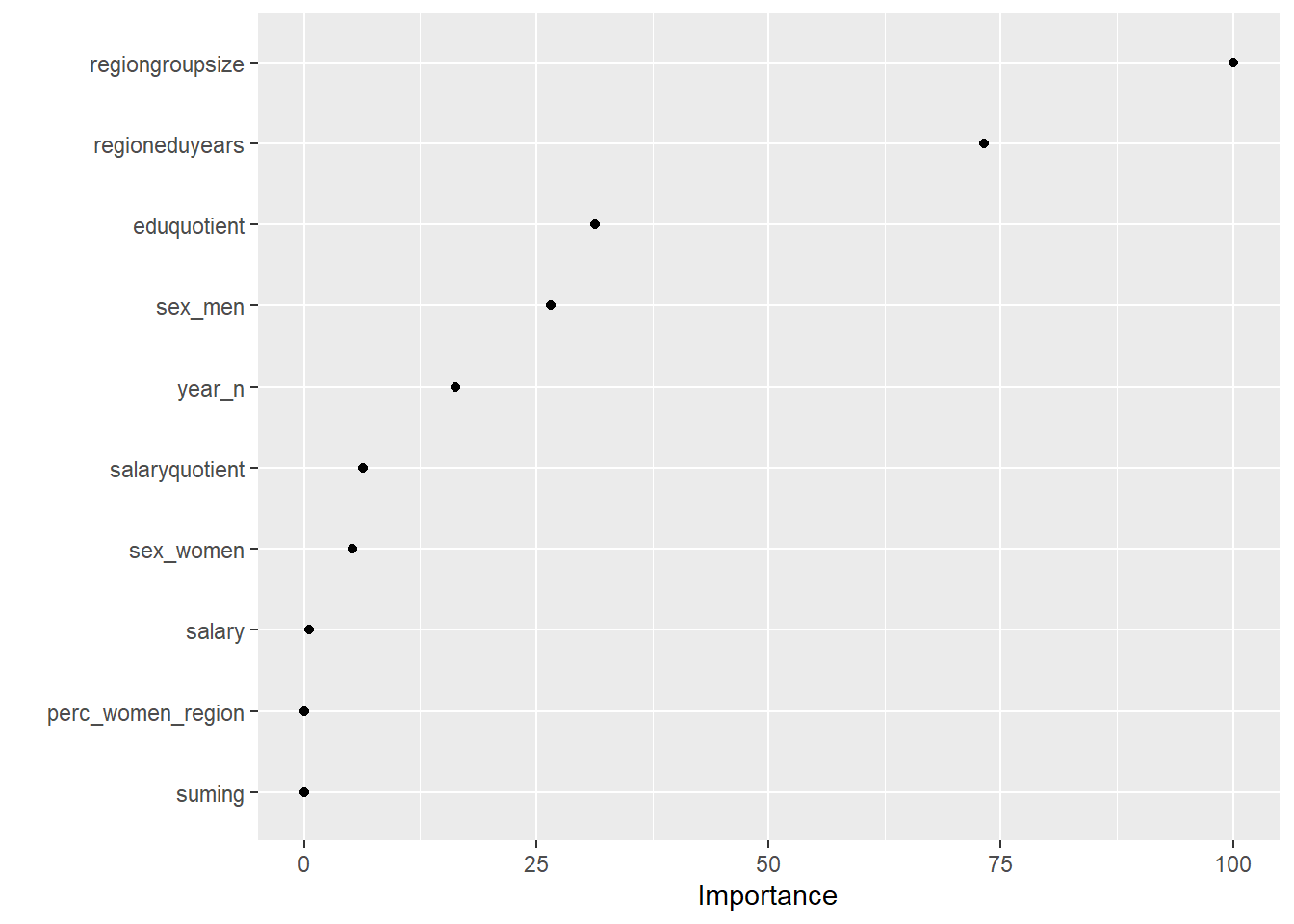Figure 1: Elastic net search on the data using no interactions, Year 2014 - 2018

tbnum_glmnet$bestTune ## alpha lambda ## 349 0.9052632 0.0002658605 elastic_min <- glmnet( x = X, y = Y, alpha = .1 ) plot(elastic_min, xvar = "lambda", main = "Elastic net penalty\n\n")Figure 2: Elastic net search on the data using no interactions, Year 2014 - 2018 I use MARS to fit the best signals using from the elastic net using no interactions. Four predictors minimise the AIC while still ensuring that the coefficients are valid, testing them with bootstrap. temp <- dplyr::select(tbnum, c(perc_women_eng_region, regiongroupsize, regioneduyears, eduquotient, sex_men)) mmod_scaled <- earth(perc_women_eng_region ~ ., weights = tbnum_weights, data = temp, nk = 9, degree = 1) summary (mmod_scaled) ## Call: earth(formula=perc_women_eng_region~., data=temp, weights=tbnum_weights, ## degree=1, nk=9) ## ## coefficients ## (Intercept) 0.188579073 ## sex_men 0.020170708 ## h(0.651904-regiongroupsize) -0.017635288 ## h(regiongroupsize-0.651904) 0.032588077 ## h(-0.259811-regioneduyears) -0.021971561 ## h(regioneduyears- -0.259811) 0.021625566 ## h(-1.22297-eduquotient) -0.049922858 ## h(eduquotient- -1.22297) 0.009477773 ## ## Selected 8 of 8 terms, and 4 of 4 predictors ## Termination condition: Reached nk 9 ## Importance: regiongroupsize, regioneduyears, eduquotient, sex_men ## Weights: 21400, 6800, 11500, 3000, 2400, 500, 7000, 1900, 16000, 4100, 3... ## Number of terms at each degree of interaction: 1 7 (additive model) ## GCV 0.8258677 RSS 40.43492 GRSq 0.8250241 RSq 0.8842515 plot (mmod_scaled)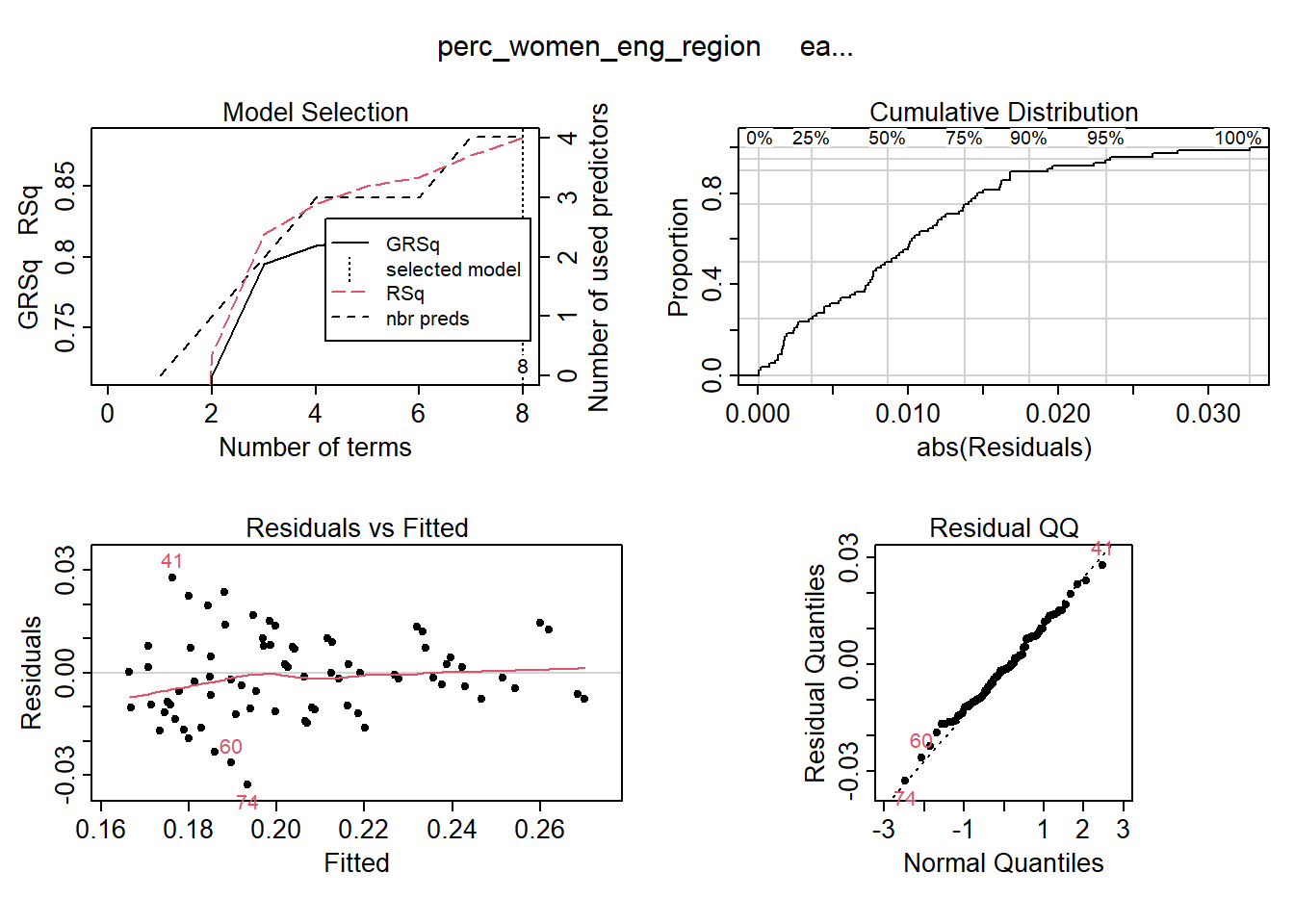Figure 3: Hockey-stick functions fit with MARS for the predictors using no interactions, Year 2014 - 2018 plotmo (mmod_scaled) ## plotmo grid: regiongroupsize regioneduyears eduquotient sex_men ## 0.2593006 -0.1394781 0 0.5Figure 4: Hockey-stick functions fit with MARS for the predictors using no interactions, Year 2014 - 2018 model_mmod_scale <- lm (perc_women_eng_region ~ sex_men + lspline(regiongroupsize, c(0.651904)) + lspline(regioneduyears, c(-0.259811)) + lspline(eduquotient, c(-1.22297)), weights = tbnum_weights, data = tbnum) model_mmod_scale <- lm (perc_women_eng_region ~ ., weights = tbnum_weights, data = tbnum) b <- regsubsets(perc_women_eng_region ~ sex_men + lspline(regiongroupsize, c(0.651904)) + lspline(regioneduyears, c(-0.259811)) + lspline(eduquotient, c(-1.22297)), data = tbnum, weights = tbnum_weights, nvmax = 12) rs <- summary(b) AIC <- 50 * log (rs$rss / 50) + (2:8) * 2
which.min (AIC)
##  7
names (rs$which[7,])[rs$which[7,]]
##  "(Intercept)"
##  "sex_men"
##  "lspline(regiongroupsize, c(0.651904))1"
##  "lspline(regiongroupsize, c(0.651904))2"
##  "lspline(regioneduyears, c(-0.259811))1"
##  "lspline(regioneduyears, c(-0.259811))2"
##  "lspline(eduquotient, c(-1.22297))1"
##  "lspline(eduquotient, c(-1.22297))2"
model_mmod_scale <- lm (perc_women_eng_region ~
sex_men +
lspline(regiongroupsize, c(0.651904)) +
lspline(regioneduyears, c(-0.259811)) +
lspline(eduquotient, c(-1.22297)),
weights = tbnum_weights,
data = tbnum)

summary (model_mmod_scale)$adj.r.squared ##  0.8723362 AIC(model_mmod_scale) ##  -426.1528 set.seed(123) results <- boot(data = tbnum, statistic = bs, R = 1000, formula = as.formula(model_mmod_scale)) #conf = coefficient not passing through zero summary (model_mmod_scale) %>% tidy() %>% mutate(bootest = tidy(results)$statistic,
bootbias = tidy(results)$bias, booterr = tidy(results)$std.error,
conf = !((tidy(confint(results))$X2.5.. < 0) & (tidy(confint(results))$X97.5.. > 0)))
## Warning in norm.inter(t, adj.alpha): extreme order statistics used as endpoints
## Warning: 'tidy.matrix' is deprecated.
## See help("Deprecated")
## Warning in norm.inter(t, adj.alpha): extreme order statistics used as endpoints
## Warning: 'tidy.matrix' is deprecated.
## See help("Deprecated")
## # A tibble: 8 x 9
##   term      estimate std.error statistic  p.value bootest bootbias booterr conf
##   <chr>        <dbl>     <dbl>     <dbl>    <dbl>   <dbl>    <dbl>   <dbl> <lgl>
## 1 (Interce~  0.244     0.0177      13.8  2.05e-21 0.244    4.73e-3 0.0376  TRUE
## 2 sex_men    0.0202    0.00509      3.96 1.82e- 4 0.0202   2.44e-3 0.00694 TRUE
## 3 lspline(~  0.0176    0.00437      4.03 1.42e- 4 0.0176   9.52e-4 0.00560 TRUE
## 4 lspline(~  0.0326    0.00828      3.94 1.97e- 4 0.0326  -2.36e-3 0.0135  TRUE
## 5 lspline(~  0.0220    0.00466      4.72 1.23e- 5 0.0220  -3.76e-3 0.00672 TRUE
## 6 lspline(~  0.0216    0.00490      4.41 3.78e- 5 0.0216   4.59e-4 0.00672 TRUE
## 7 lspline(~  0.0499    0.0133       3.76 3.56e- 4 0.0499   3.70e-3 0.0293  TRUE
## 8 lspline(~  0.00948   0.00357      2.66 9.85e- 3 0.00948 -3.47e-3 0.00491 TRUE
plot(results, index=1) # intercept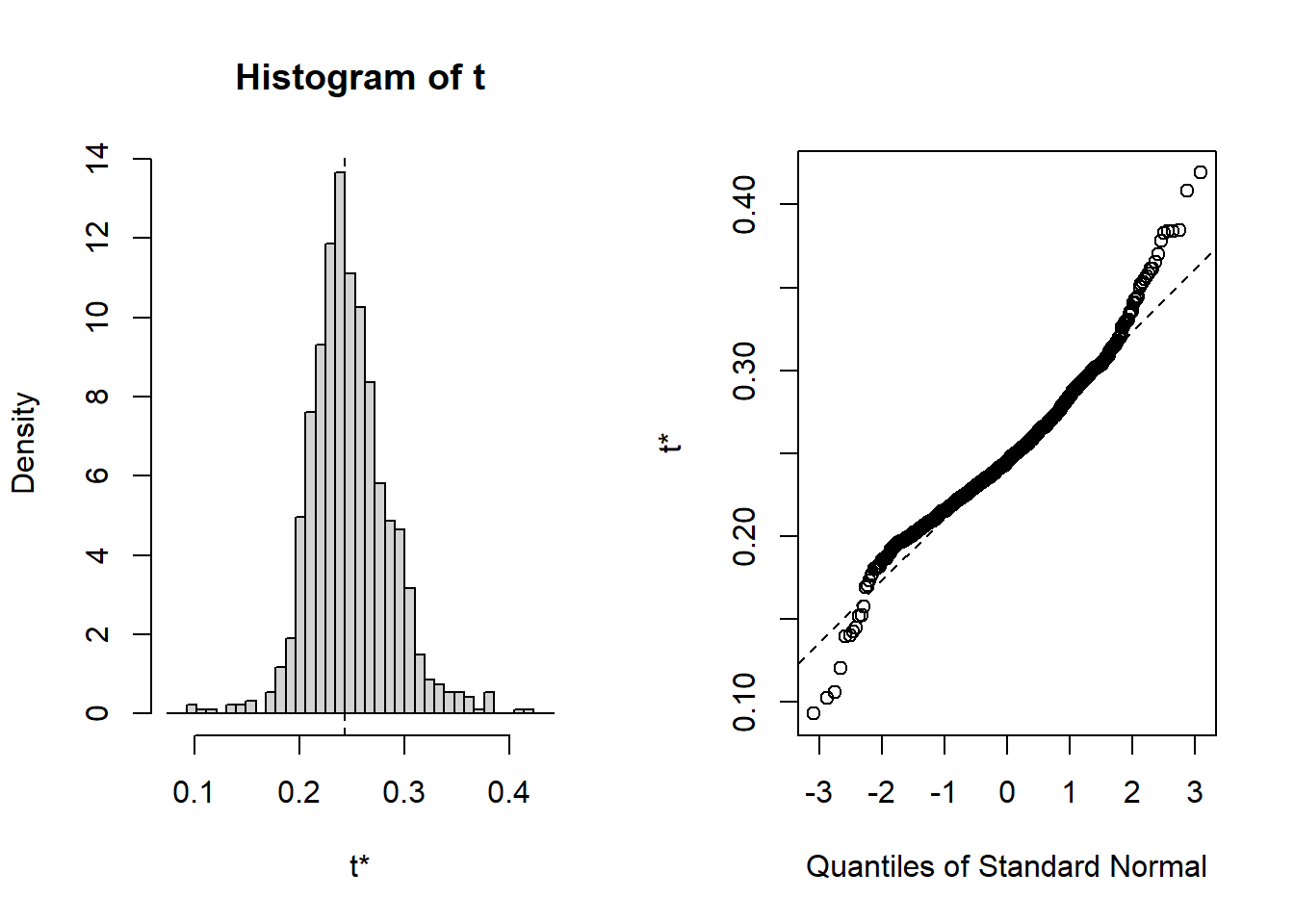Figure 5: Hockey-stick functions fit with MARS for the predictors using no interactions, Year 2014 - 2018

plot_model will show diagnostics for the model, a residual plot, scale location plot, residual density and the regression error characteristic curve. It will also show the residuals versus the actual response to help find outliers. It will plot the feature importance and feature effects. In addition, it will plot how strongly features interact with each other and the 2-way interactions with regiongroupsize and all other features. The interaction measure regards how much of the variance of f(x) is explained by the interaction. The measure is between 0 (no interaction) and 1 (= 100% of variance of f(x) due to interactions). Regiongroupsize is of special interest since it is the feature with the strongest importance to the per cent of engineers who are women in the region.

plot_model <- function(model){
invisible(capture.output(exp_model <- DALEX::explain(model, data = tbnum, y = tbnum$perc_women_eng_region))) # Knit and DALEX::explain generates invalid rss feed lm_mr <- model_residual(exp_model) predictor <- Predictor$new(model,
data = dplyr::select(tbnum, -perc_women_eng_region),
y = tbnum$perc_women_eng_region) writeLines("") print(paste ("Model RMSE: ", rmse(predict(model), tbnum$perc_women_eng_region)))

p1 <- plot(lm_mr, type = "residual")

p2 <- plot(lm_mr, type = "scalelocation")

p3 <- plot(lm_mr, type = "residual_density")

p4 <- plot(lm_mr, type = "rec")

print(gridExtra::grid.arrange(p1, p2, p3, p4, ncol = 2))

print(plot_residual(lm_mr, variable = "_y_hat_", nlabel = 10))

print(plot (FeatureImp$new(predictor, loss = "mae"))) print(plot (FeatureEffects$new(predictor)))

p1 <- plot (Interaction$new(predictor)) p2 <- plot (Interaction$new(predictor, feature = "regiongroupsize"))

print(gridExtra::grid.arrange(p1, p2, ncol = 2))
}

Manual Data fit with Regularized Regression and MARS

model <- lm (
perc_women_eng_region ~
sex_men +
lspline(regiongroupsize, c(0.651904)) +
lspline(regioneduyears, c(-0.259811)) +
lspline(eduquotient, c(-1.22297)),
weights = tbnum_weights,
data = tbnum)

plot_model(model)
##
##  "Model RMSE:  0.0119381601517971"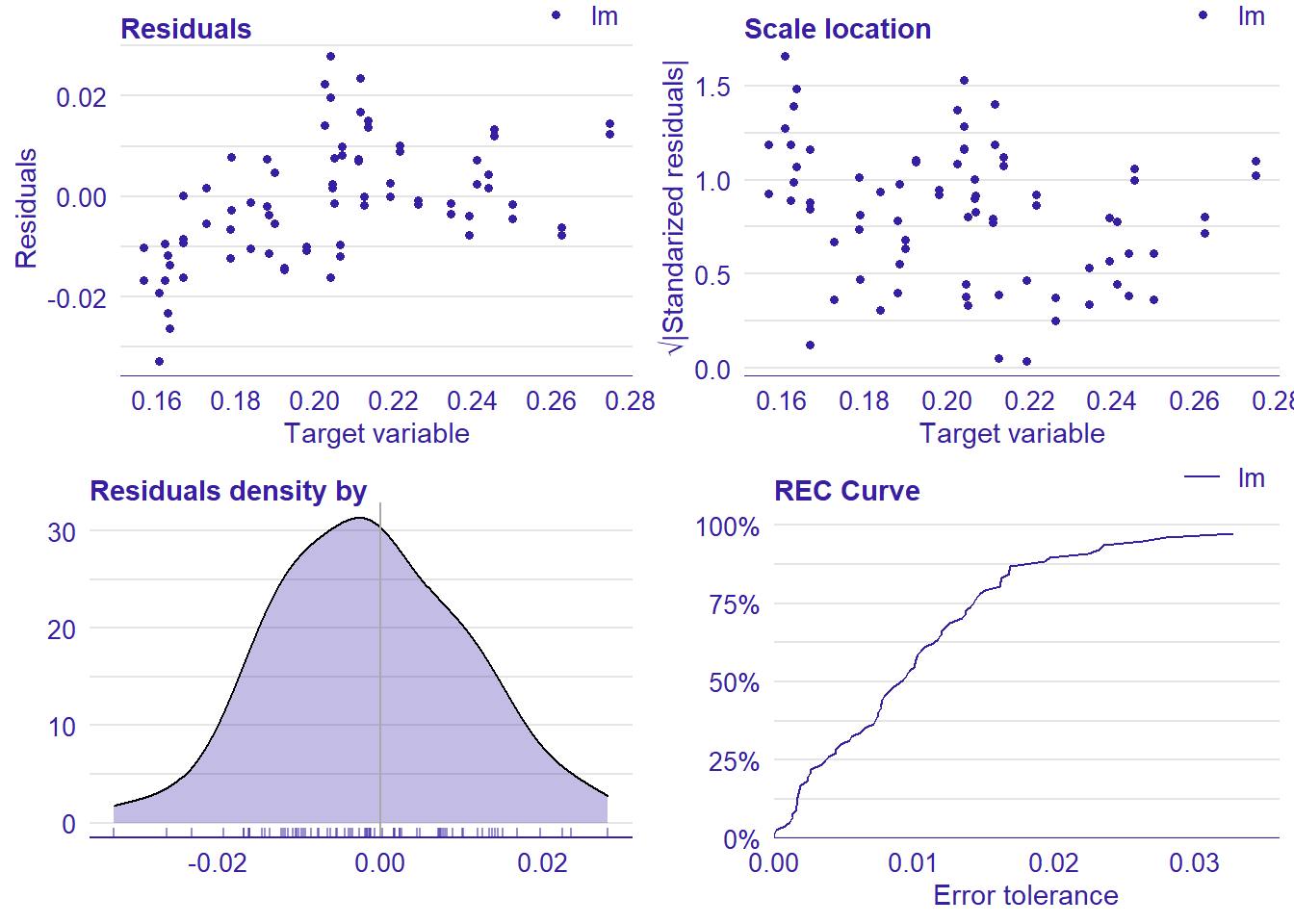Figure 6: Manual Data fit with Regularized Regression and MARS, Year 2014 - 2018

## TableGrob (2 x 2) "arrange": 4 grobs
##   z     cells    name           grob
## 1 1 (1-1,1-1) arrange gtable[layout]
## 2 2 (1-1,2-2) arrange gtable[layout]
## 3 3 (2-2,1-1) arrange gtable[layout]
## 4 4 (2-2,2-2) arrange gtable[layout]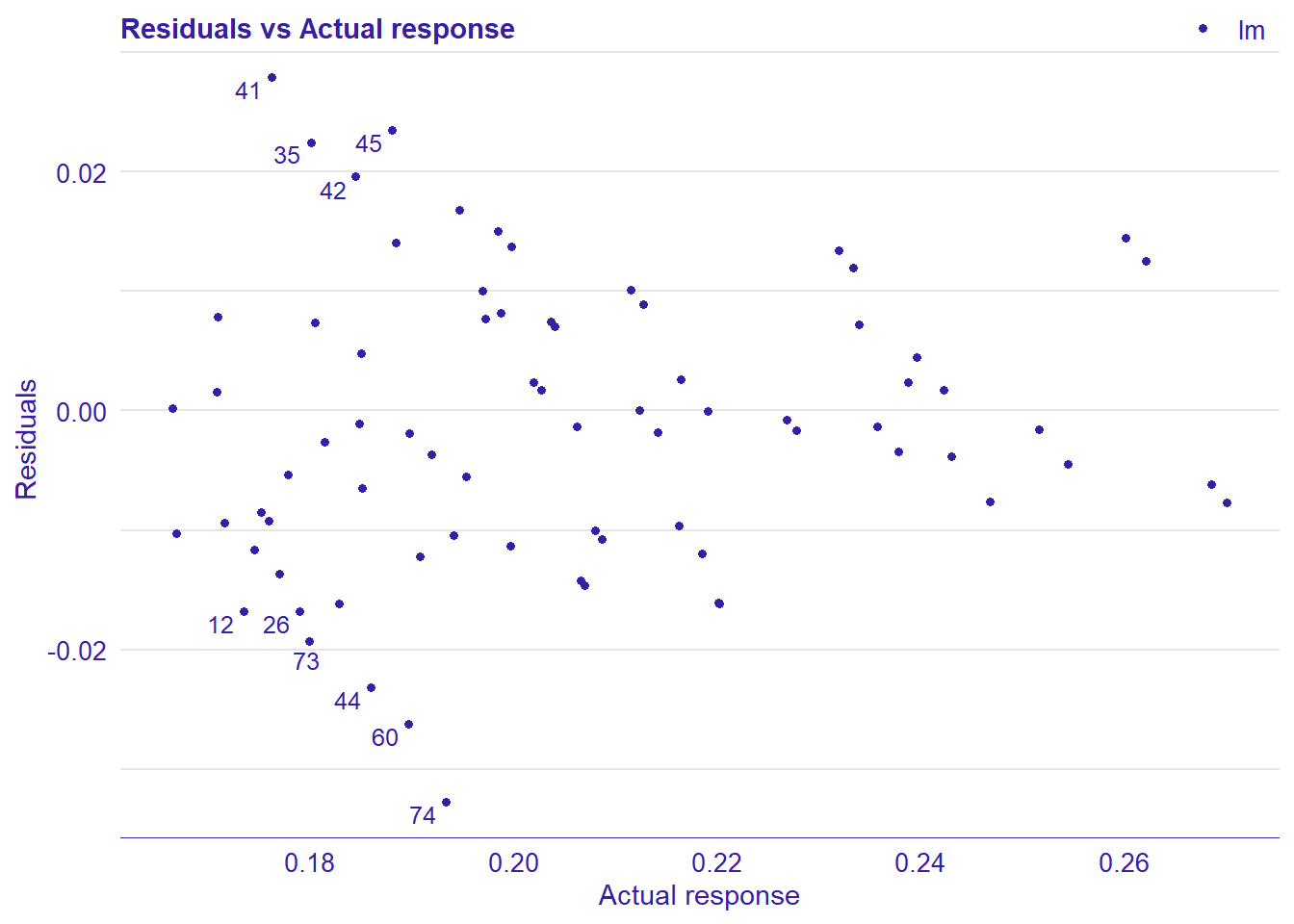Figure 7: Manual Data fit with Regularized Regression and MARS, Year 2014 - 2018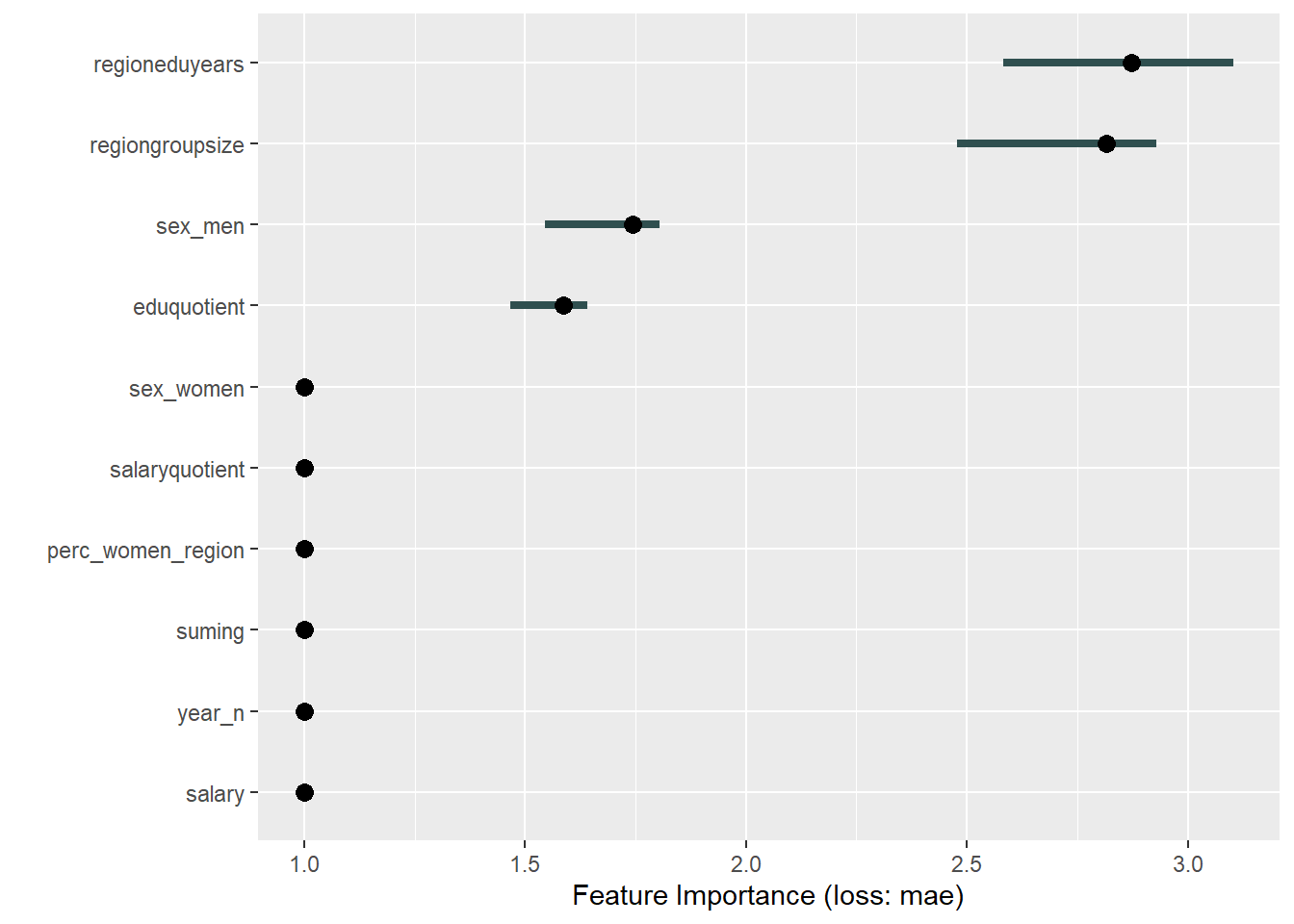Figure 8: Manual Data fit with Regularized Regression and MARS, Year 2014 - 2018

##
## Attaching package: 'patchwork'
## The following object is masked from 'package:MASS':
##
##     areaFigure 9: Manual Data fit with Regularized Regression and MARS, Year 2014 - 2018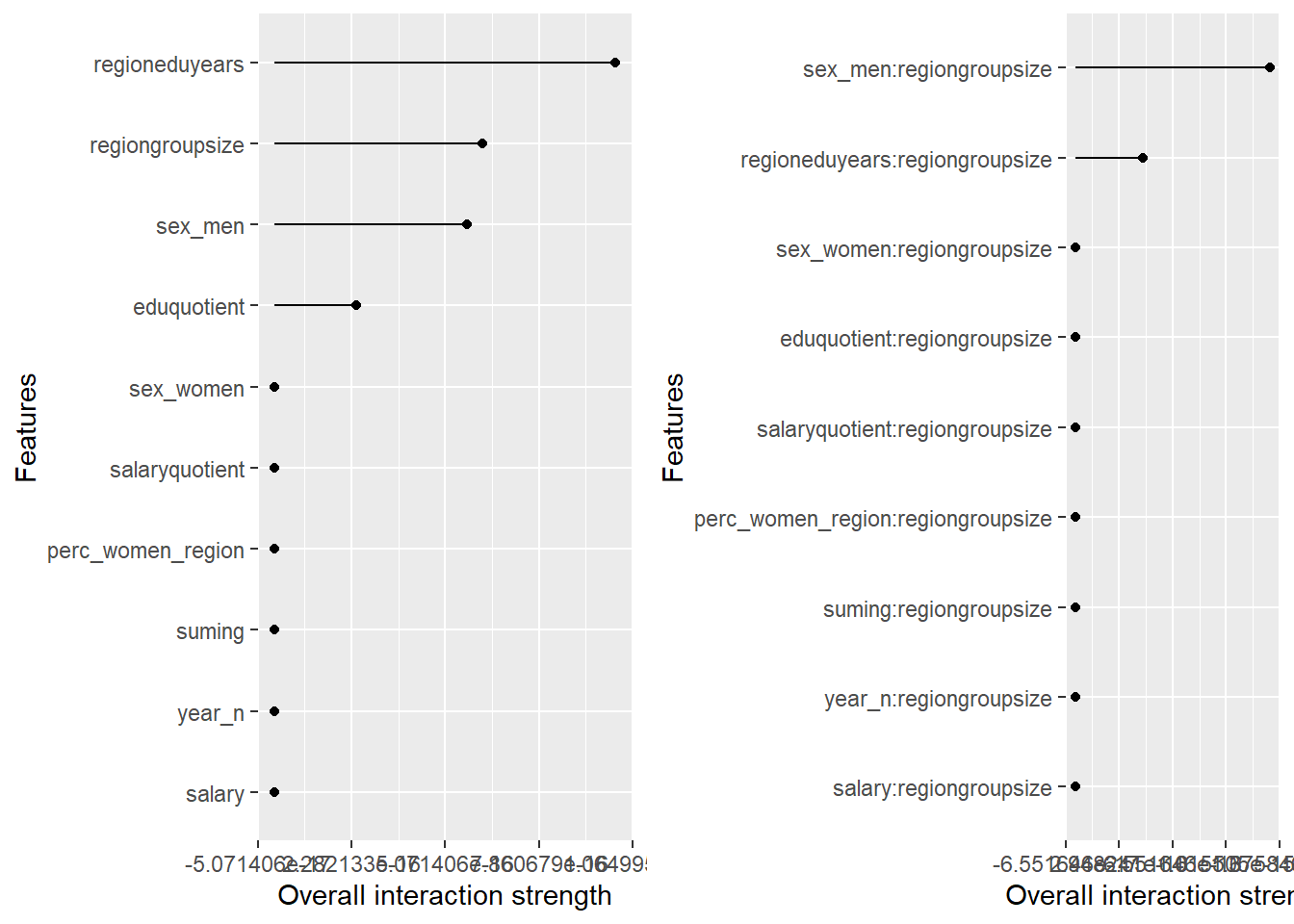Figure 10: Manual Data fit with Regularized Regression and MARS, Year 2014 - 2018

## TableGrob (1 x 2) "arrange": 2 grobs
##   z     cells    name           grob
## 1 1 (1-1,1-1) arrange gtable[layout]
## 2 2 (1-1,2-2) arrange gtable[layout]

Data fit with Regularized Regression

X <- model.matrix(perc_women_eng_region ~ ., tbnum)[, -1]

Y <- tbnum$perc_women_eng_region set.seed(123) # for reproducibility model <- caret::train( x = X, y = Y, weights = tbnum_weights, method = "glmnet", preProc = c("zv", "center", "scale"), trControl = trainControl(method = "cv", number = 10), tuneLength = 20 ) ## Warning in nominalTrainWorkflow(x = x, y = y, wts = weights, info = trainInfo, : ## There were missing values in resampled performance measures. plot_model(model) ## ##  "Model RMSE: 0.0115236920245644"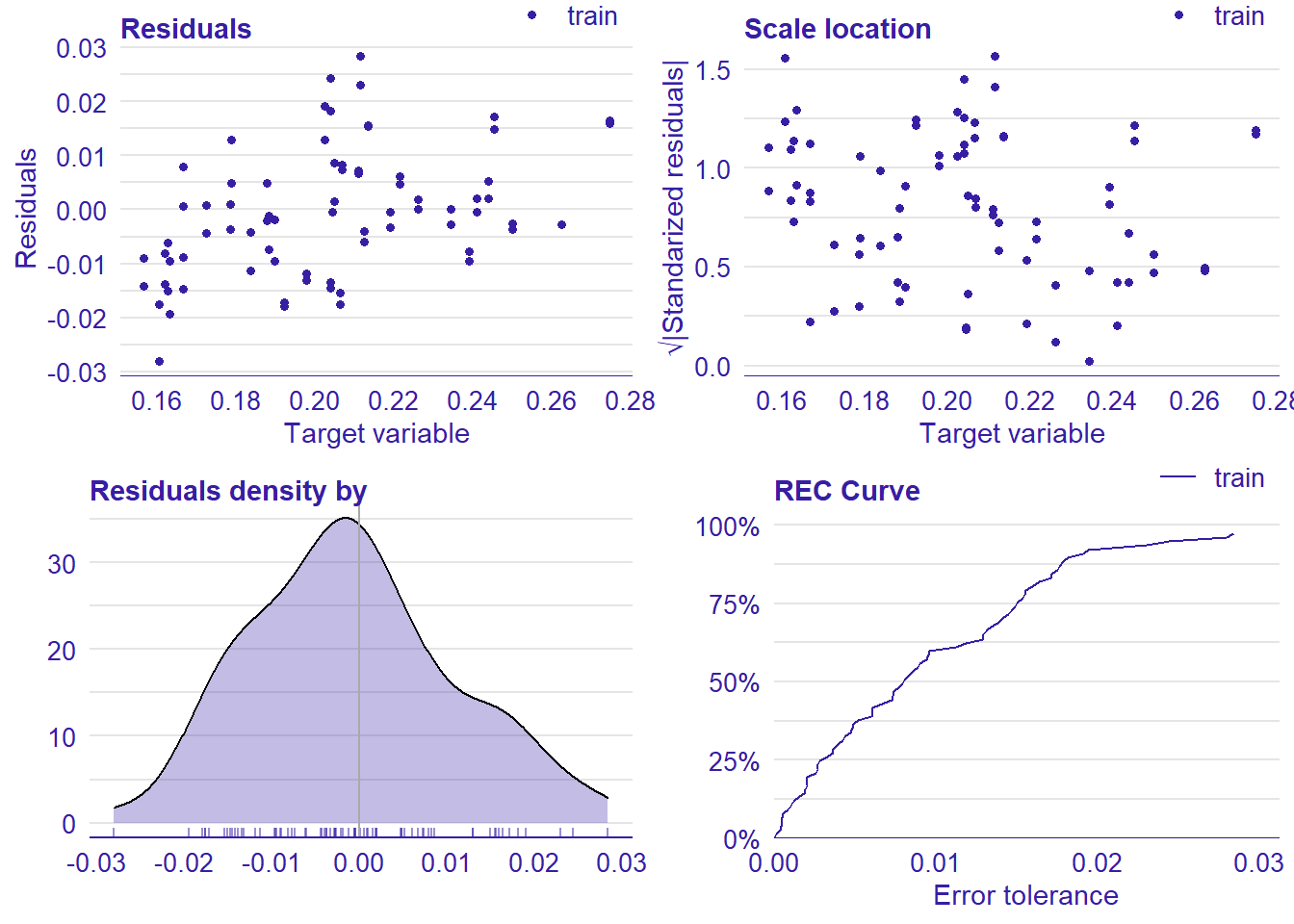Figure 11: Data fit with Regularized Regression, Year 2014 - 2018 ## TableGrob (2 x 2) "arrange": 4 grobs ## z cells name grob ## 1 1 (1-1,1-1) arrange gtable[layout] ## 2 2 (1-1,2-2) arrange gtable[layout] ## 3 3 (2-2,1-1) arrange gtable[layout] ## 4 4 (2-2,2-2) arrange gtable[layout]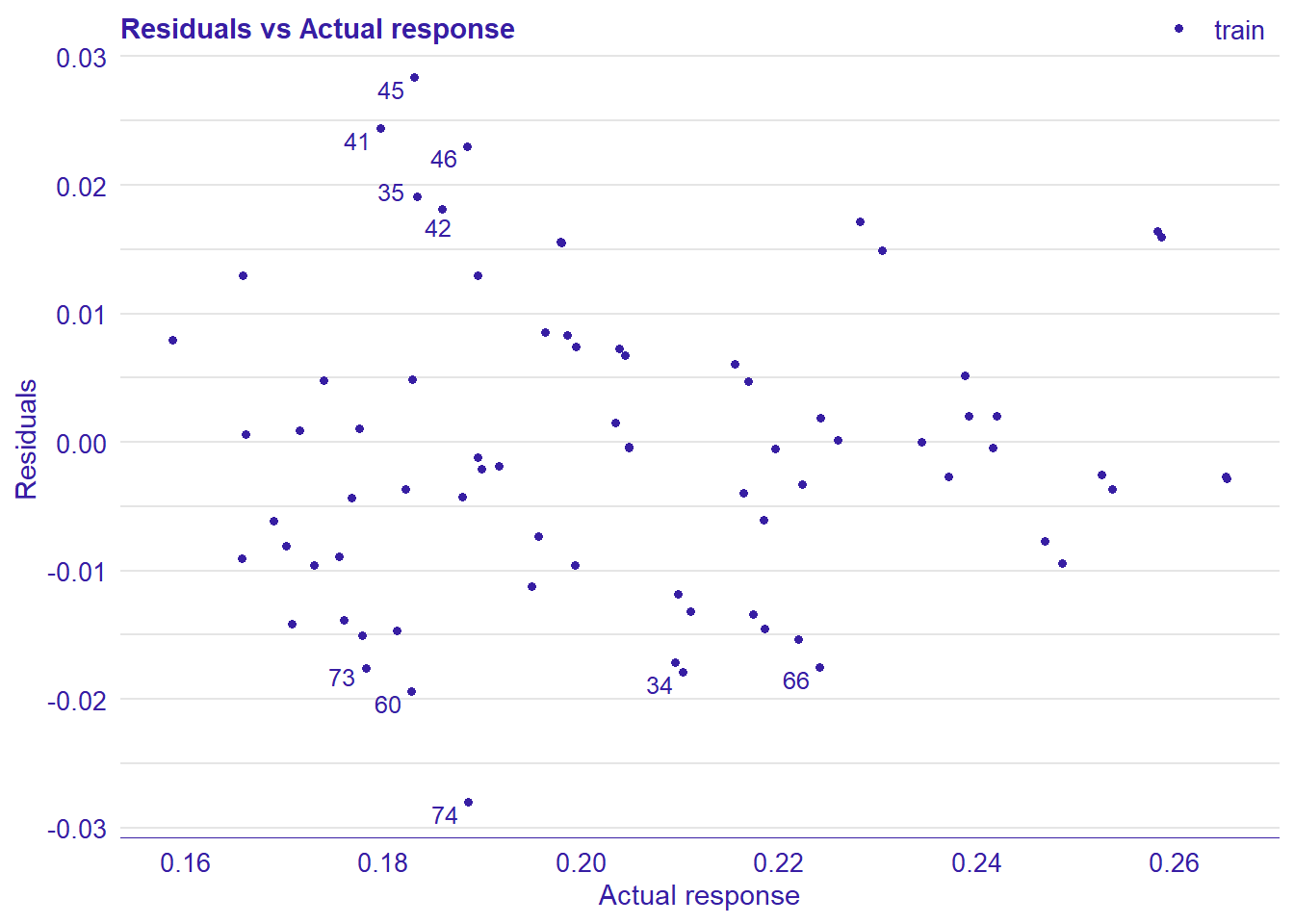Figure 12: Data fit with Regularized Regression, Year 2014 - 2018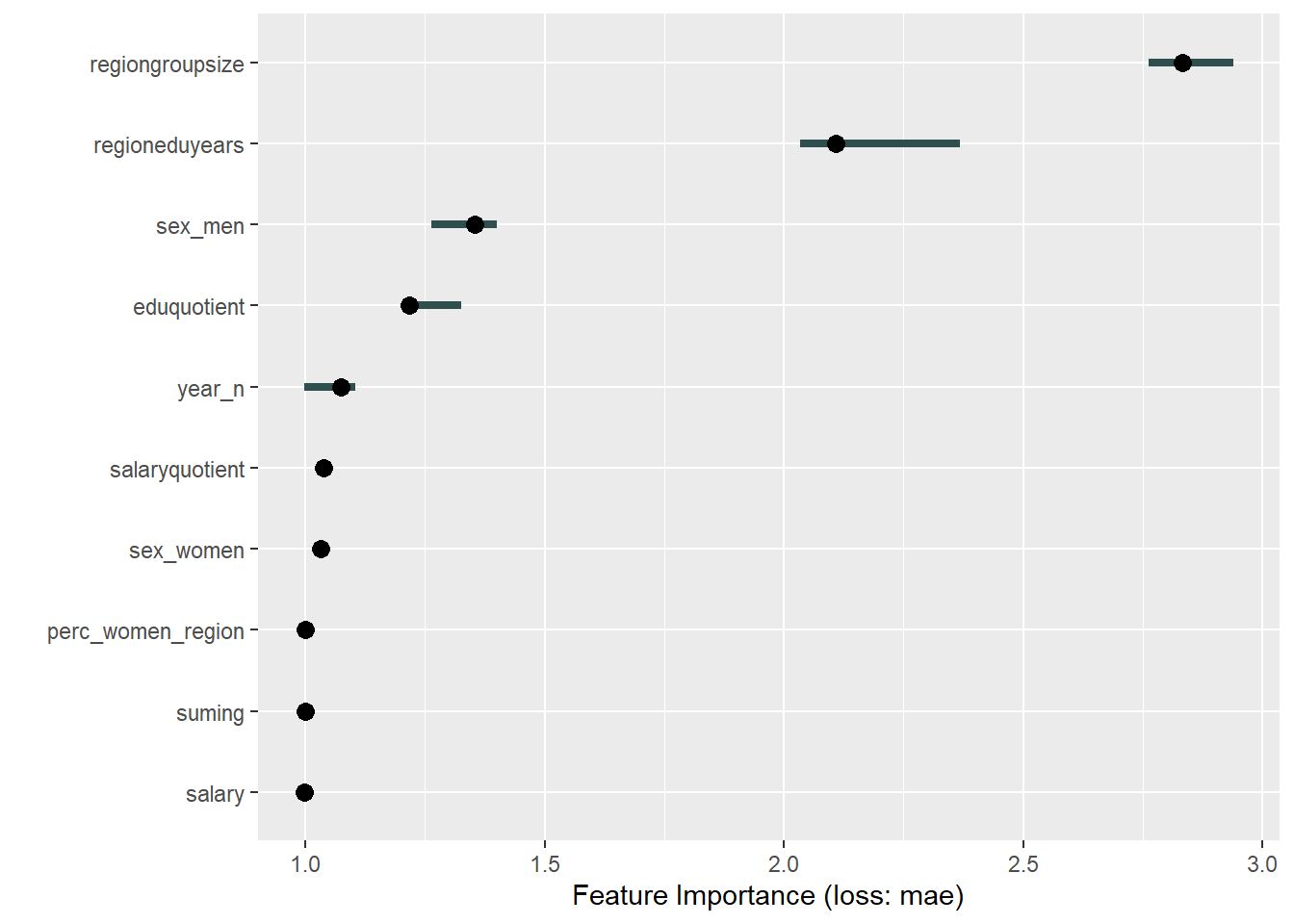Figure 13: Data fit with Regularized Regression, Year 2014 - 2018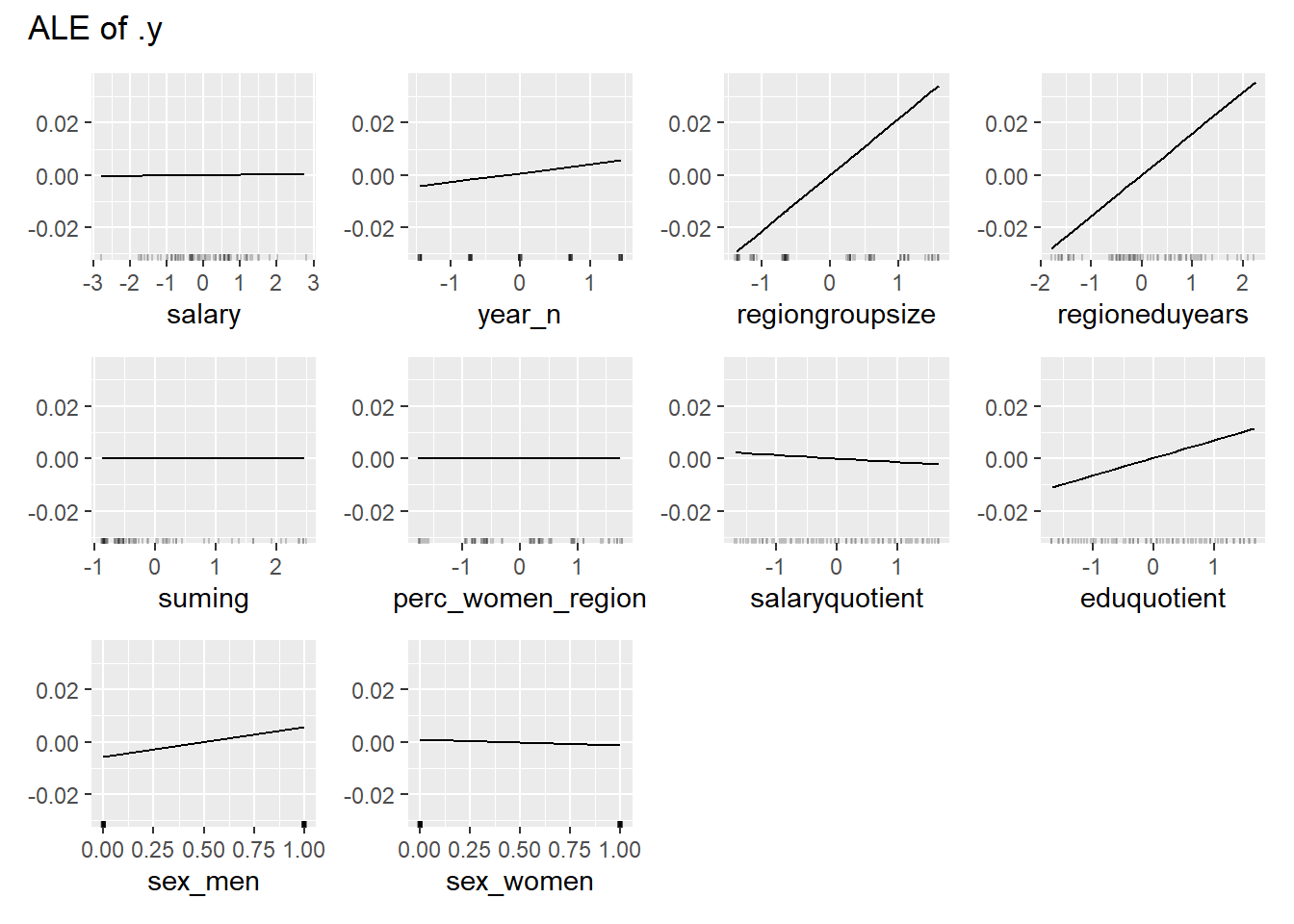Figure 14: Data fit with Regularized Regression, Year 2014 - 2018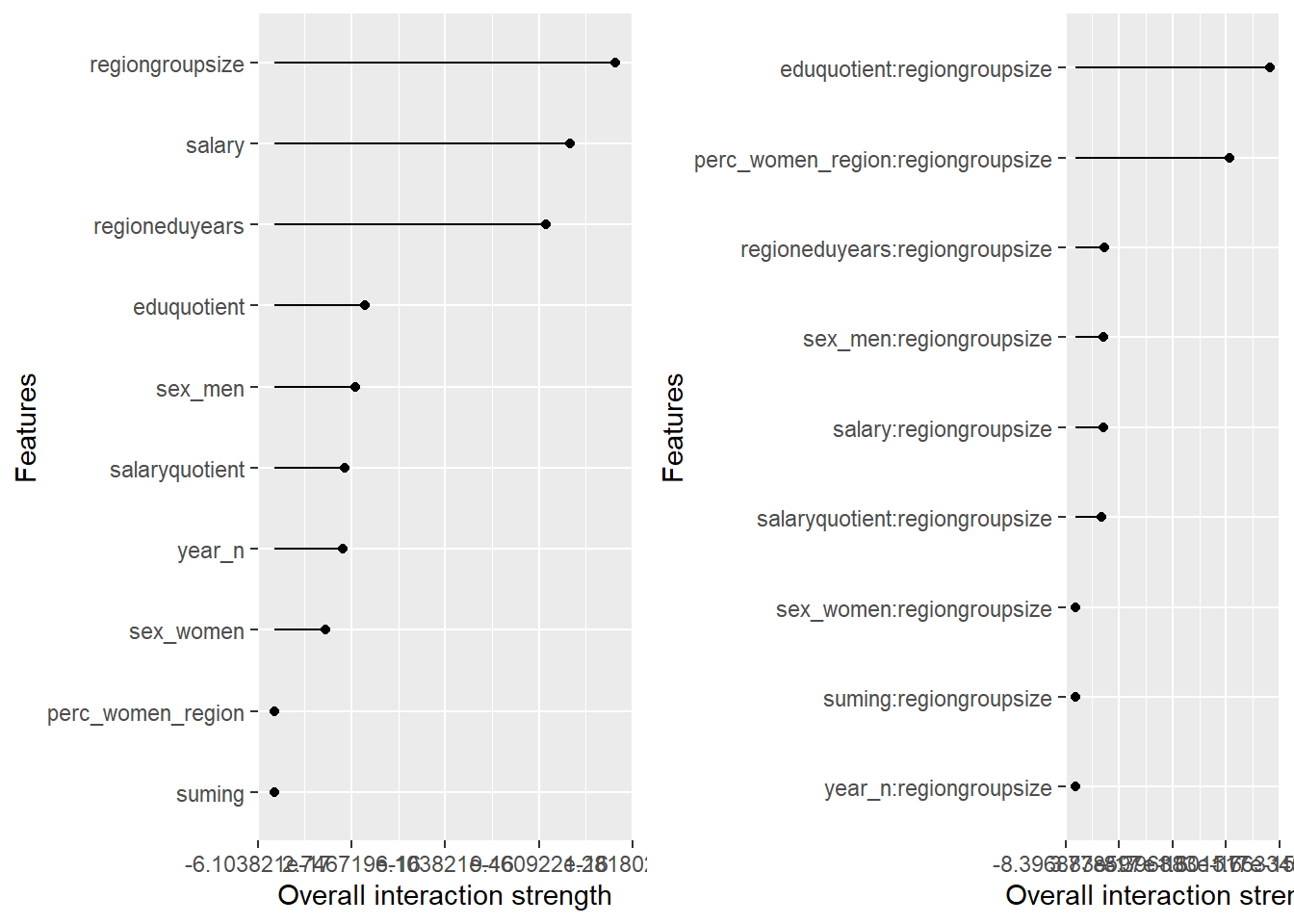Figure 15: Data fit with Regularized Regression, Year 2014 - 2018 ## TableGrob (1 x 2) "arrange": 2 grobs ## z cells name grob ## 1 1 (1-1,1-1) arrange gtable[layout] ## 2 2 (1-1,2-2) arrange gtable[layout] Data fit with Multivariate Adaptive Regression Splines hyper_grid <- expand.grid( degree = 1, nprune = seq(2, 100, length.out = 10) %>% floor() ) set.seed(123) # for reproducibility model <- caret::train( x = data.frame(subset(tbnum, select = -perc_women_eng_region)), y = tbnum$perc_women_eng_region,
method = "earth",
weights = tbnum_weights,
trControl = trainControl(method = "cv", number = 10),
tuneGrid = hyper_grid
)

plot_model(model)
## Warning in if (class(response) == "data.frame") response <- as.matrix(response):
## the condition has length > 1 and only the first element will be used

## Warning in if (class(response) == "data.frame") response <- as.matrix(response):
## the condition has length > 1 and only the first element will be used
##
##  "Model RMSE:  0.00915463662070812"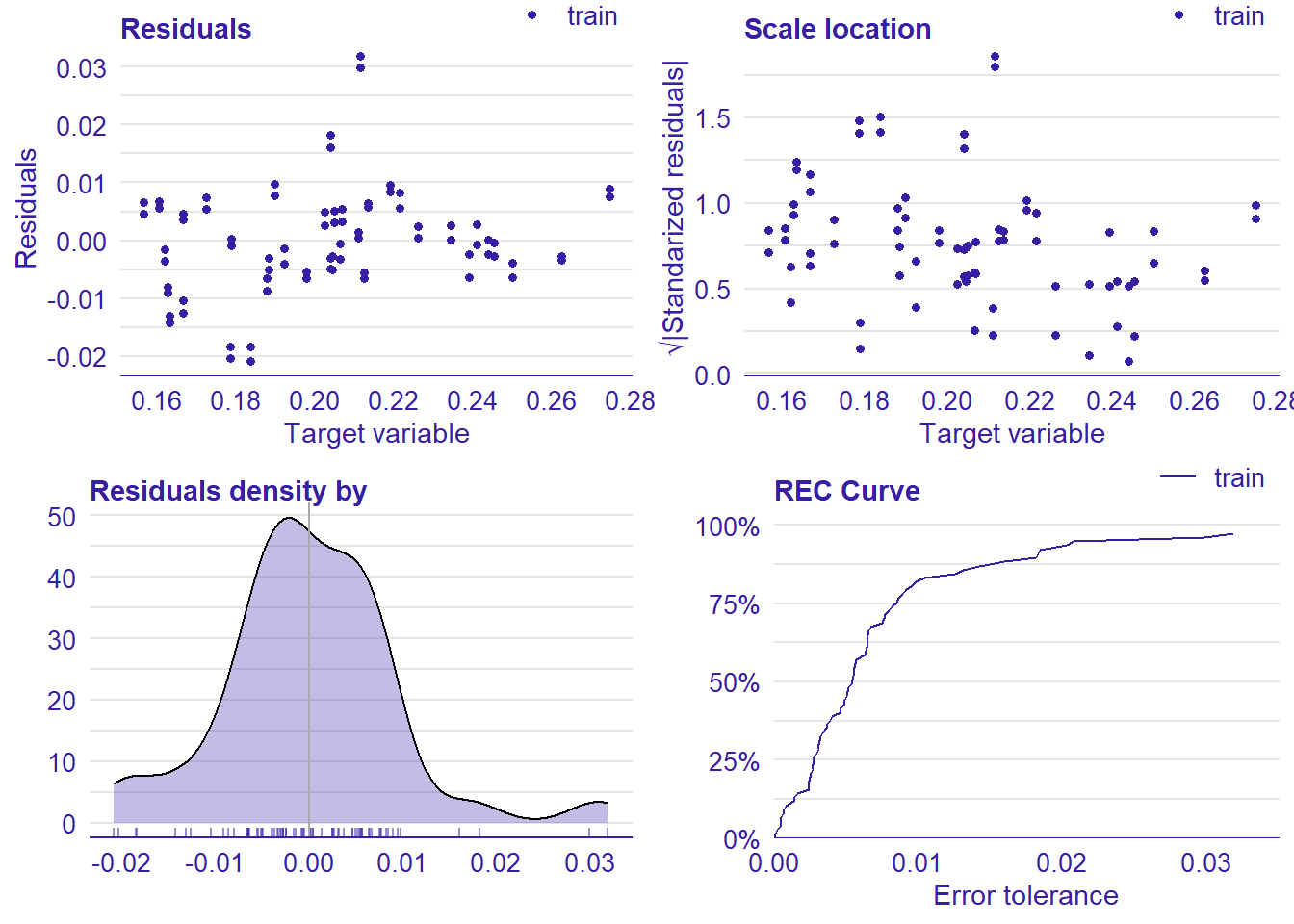Figure 16: Data fit with Multivariate Adaptive Regression Splines, Year 2014 - 2018

## TableGrob (2 x 2) "arrange": 4 grobs
##   z     cells    name           grob
## 1 1 (1-1,1-1) arrange gtable[layout]
## 2 2 (1-1,2-2) arrange gtable[layout]
## 3 3 (2-2,1-1) arrange gtable[layout]
## 4 4 (2-2,2-2) arrange gtable[layout]Figure 17: Data fit with Multivariate Adaptive Regression Splines, Year 2014 - 2018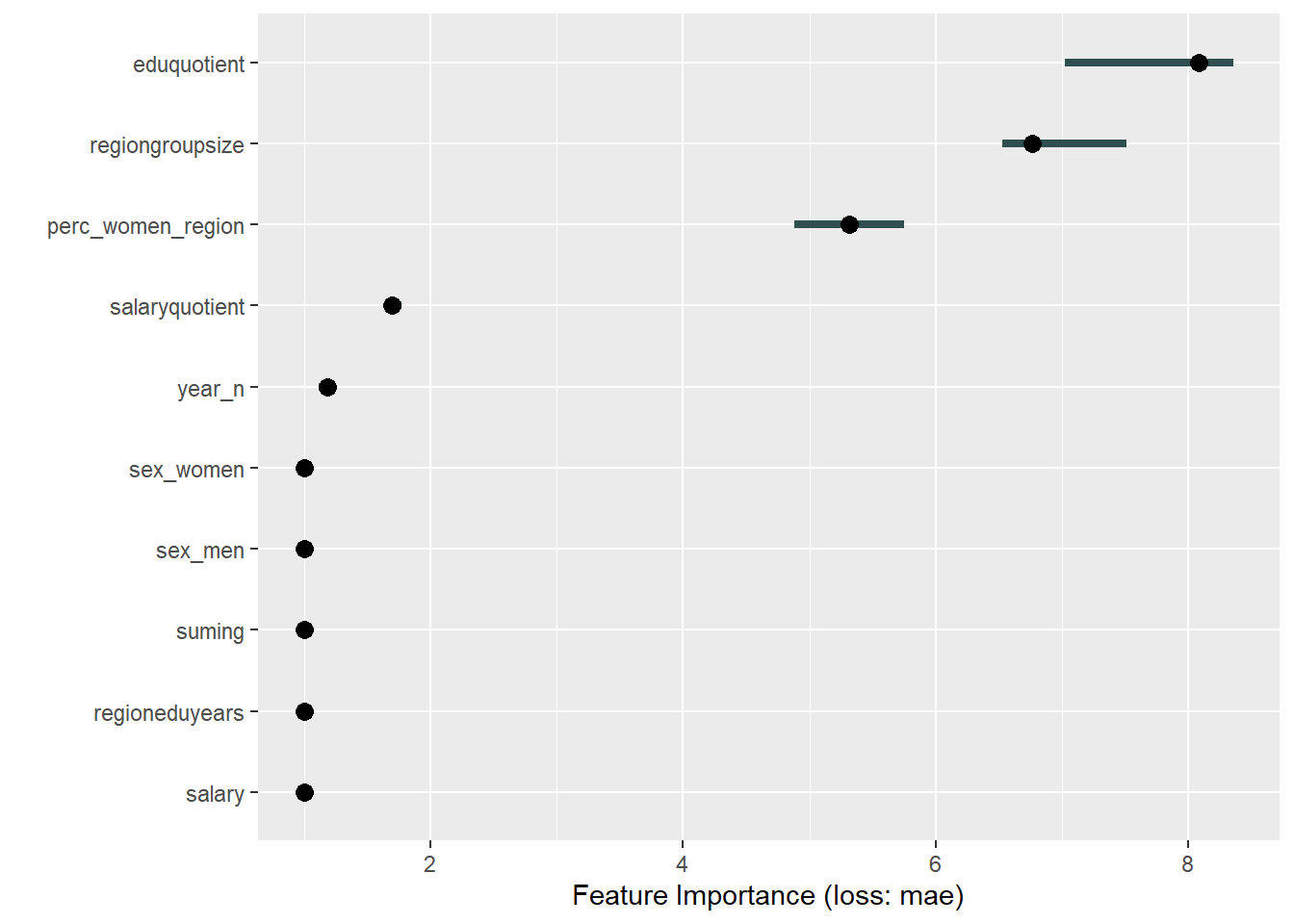Figure 18: Data fit with Multivariate Adaptive Regression Splines, Year 2014 - 2018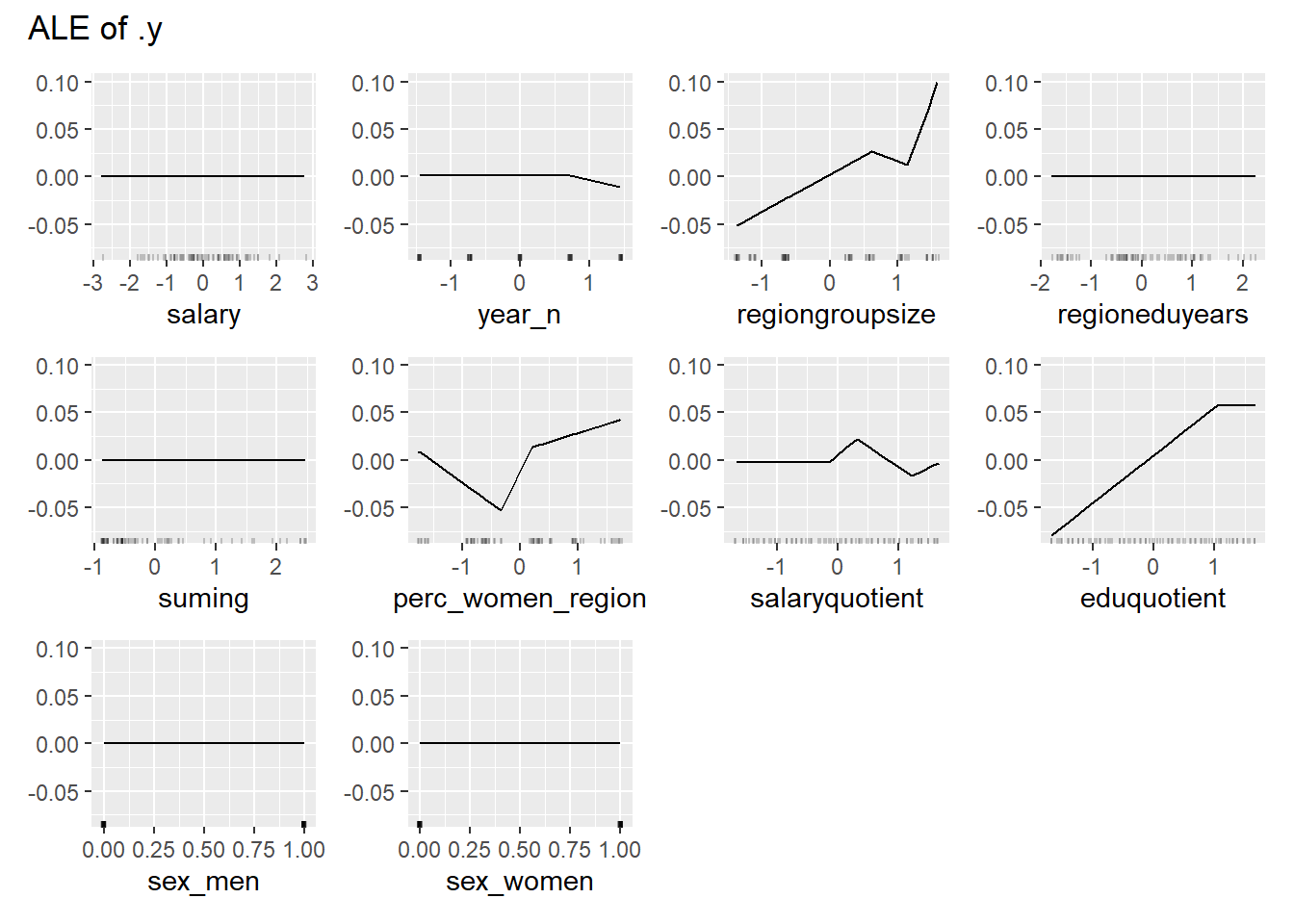Figure 19: Data fit with Multivariate Adaptive Regression Splines, Year 2014 - 2018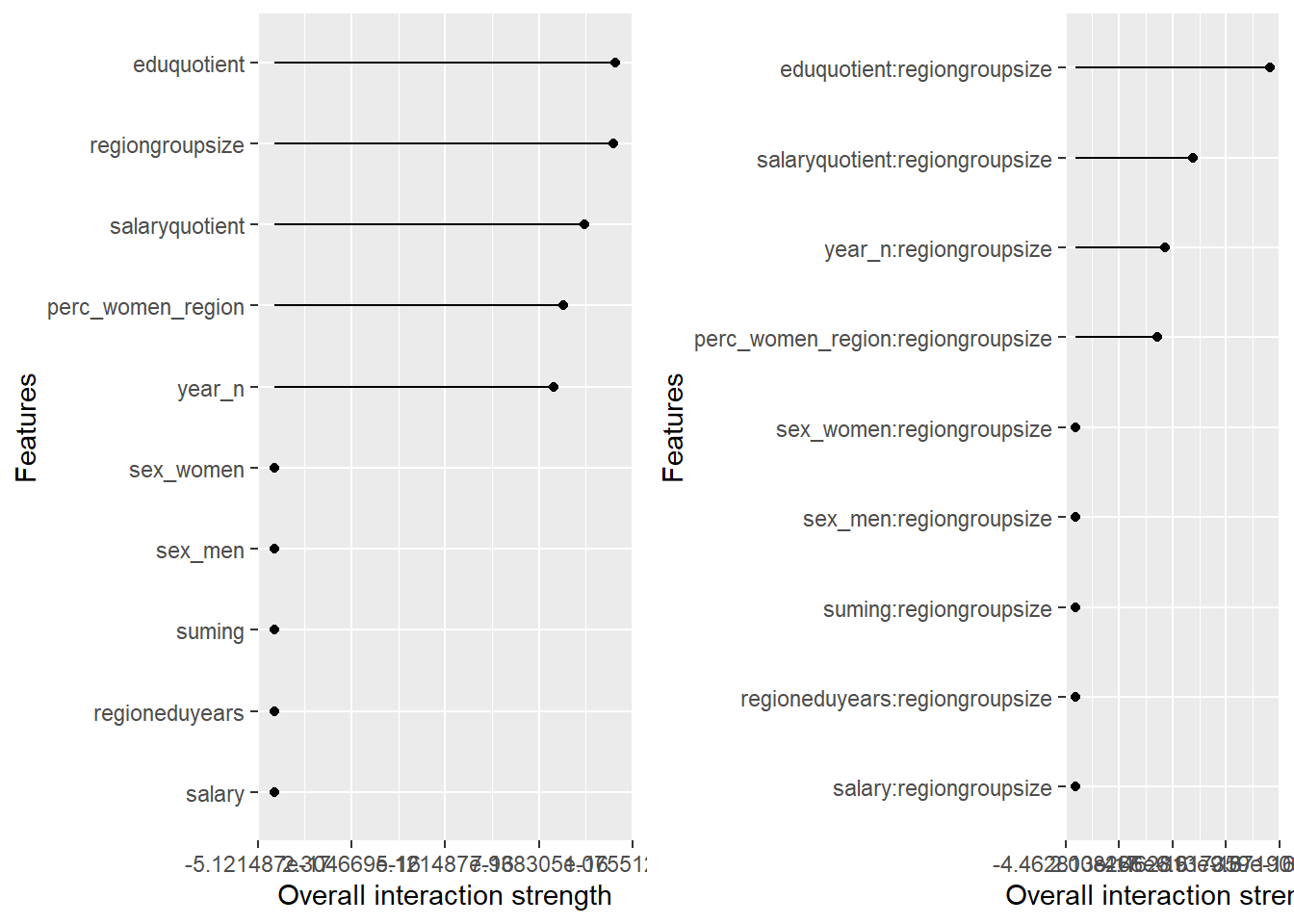Figure 20: Data fit with Multivariate Adaptive Regression Splines, Year 2014 - 2018

## TableGrob (1 x 2) "arrange": 2 grobs
##   z     cells    name           grob
## 1 1 (1-1,1-1) arrange gtable[layout]
## 2 2 (1-1,2-2) arrange gtable[layout]

Data fit with decision tree bag

set.seed(123)
model <- caret::train(
perc_women_eng_region ~ .,
data = tbnum,
method = "treebag",
weights = tbnum_weights,
trControl = trainControl(method = "cv", number = 10),
nbagg = 50,
control = rpart.control(minsplit = 2, cp = 0)
)

plot_model(model)
##
##  "Model RMSE:  0.00341044239371977"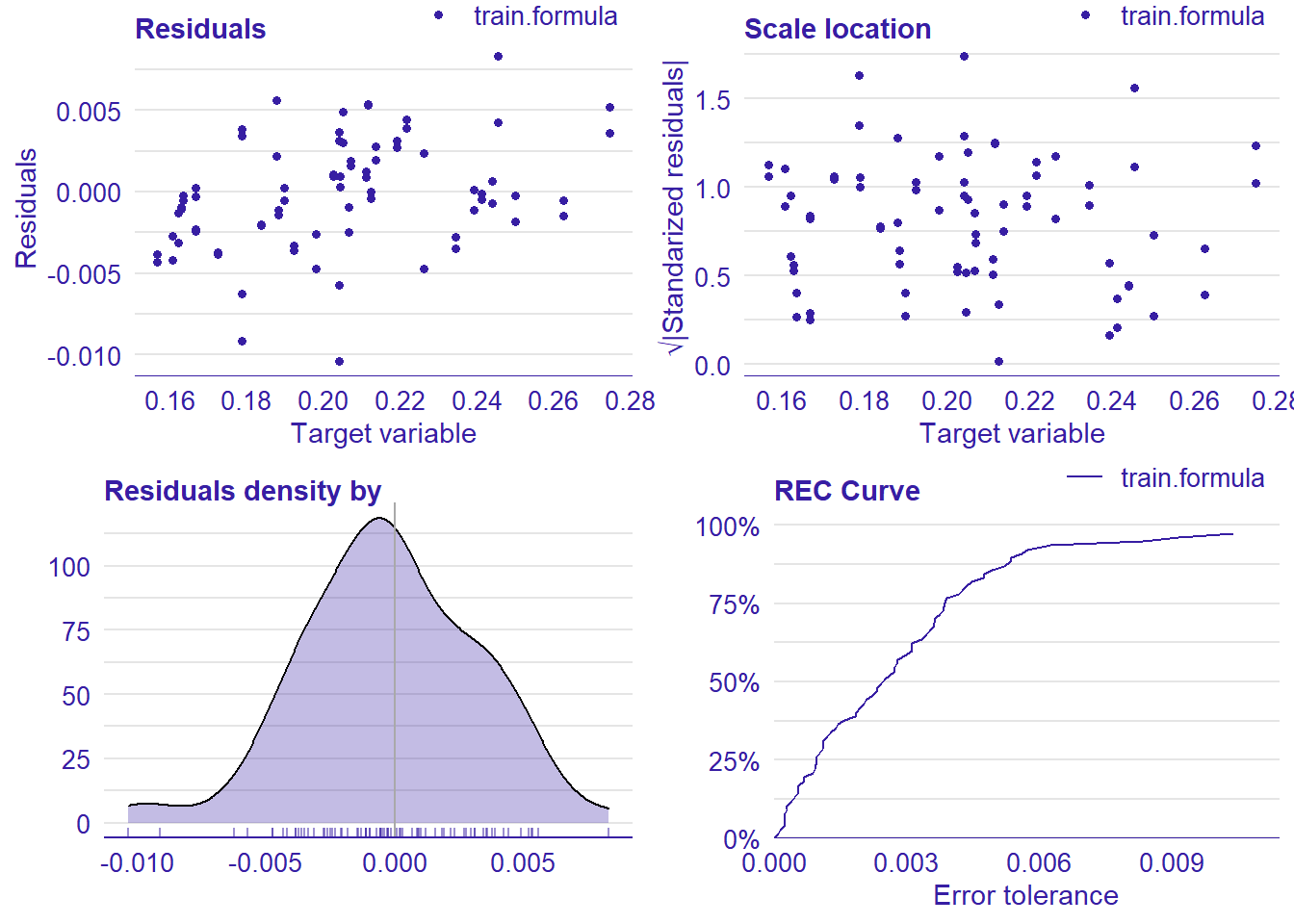Figure 21: Data fit with decision tree bag, Year 2014 - 2018

## TableGrob (2 x 2) "arrange": 4 grobs
##   z     cells    name           grob
## 1 1 (1-1,1-1) arrange gtable[layout]
## 2 2 (1-1,2-2) arrange gtable[layout]
## 3 3 (2-2,1-1) arrange gtable[layout]
## 4 4 (2-2,2-2) arrange gtable[layout]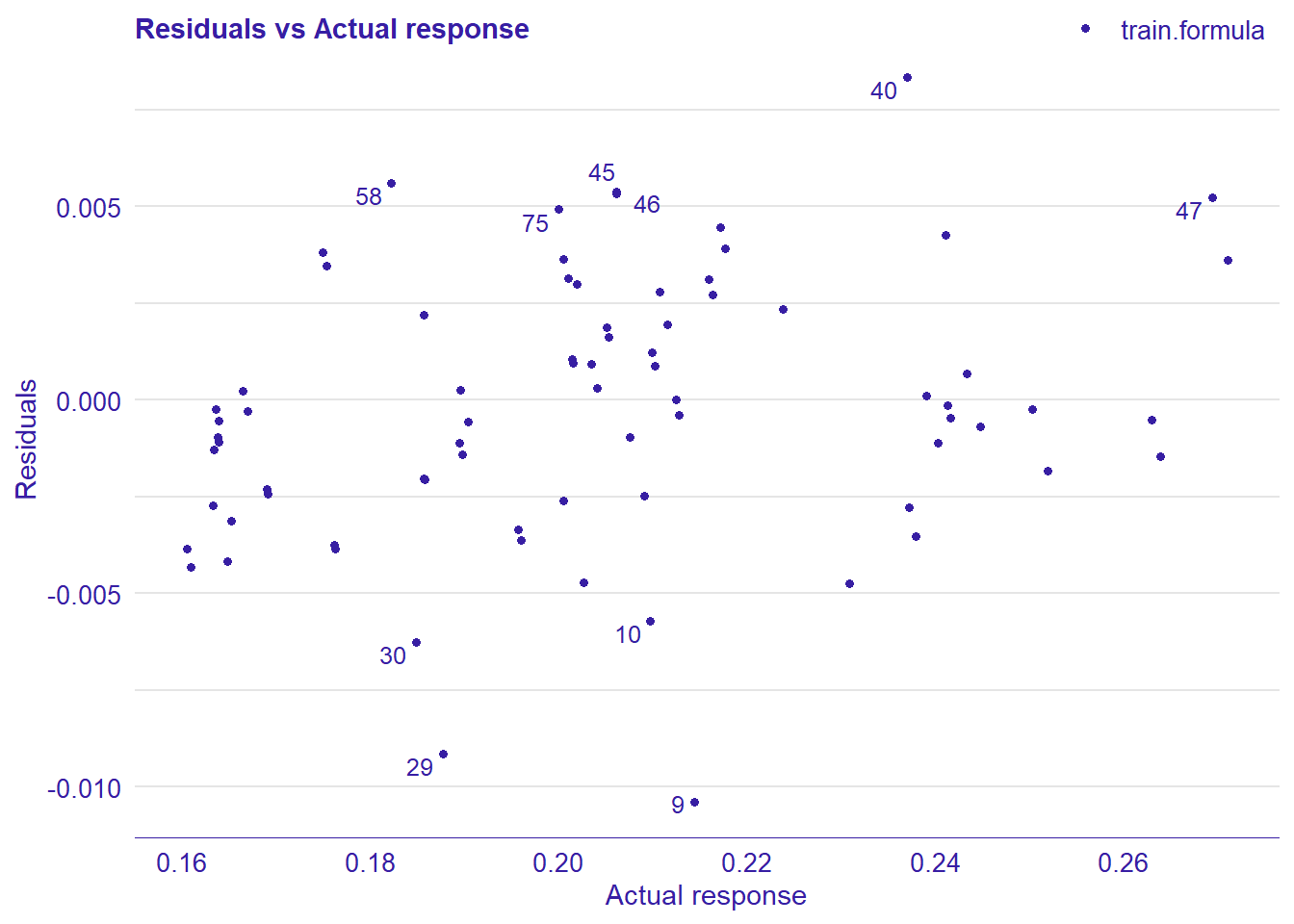Figure 22: Data fit with decision tree bag, Year 2014 - 2018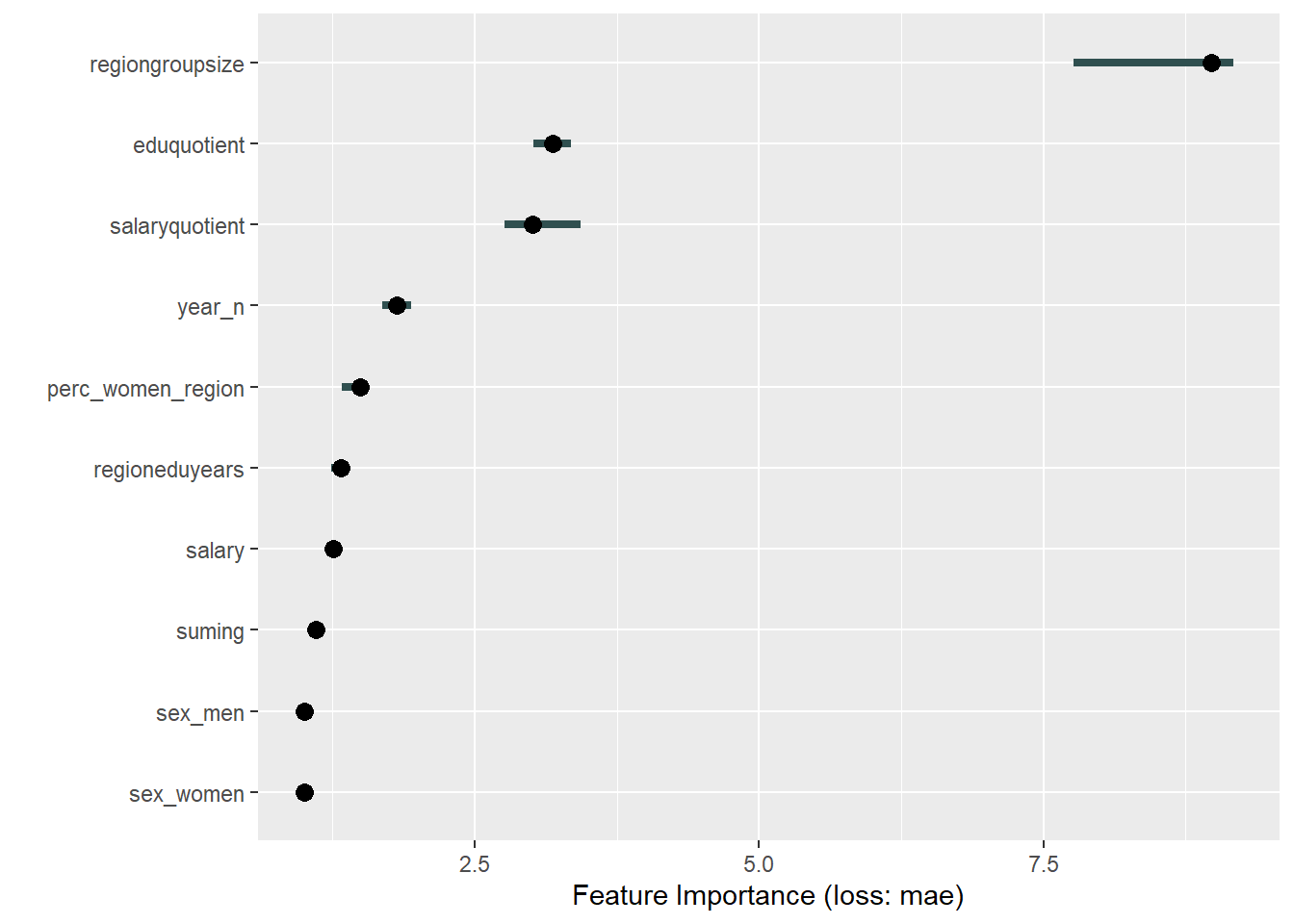Figure 23: Data fit with decision tree bag, Year 2014 - 2018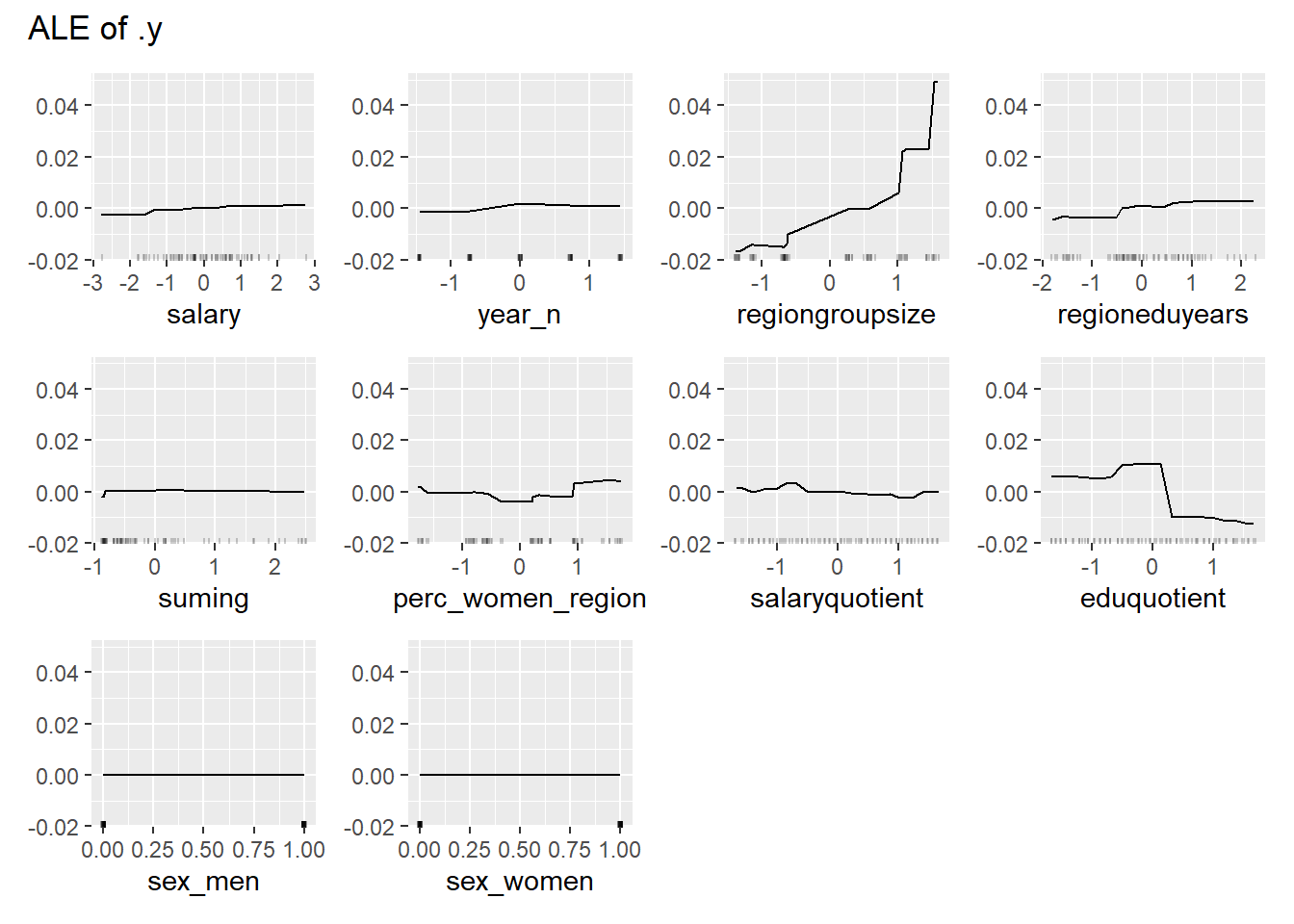Figure 24: Data fit with decision tree bag, Year 2014 - 2018Figure 25: Data fit with decision tree bag, Year 2014 - 2018

## TableGrob (1 x 2) "arrange": 2 grobs
##   z     cells    name           grob
## 1 1 (1-1,1-1) arrange gtable[layout]
## 2 2 (1-1,2-2) arrange gtable[layout]

Data fit with Random Forest

set.seed(123)
invisible(capture.output(model <- caret::train(
perc_women_eng_region  ~ .,
data = tbnum,
weights = tbnum_weights,
method = "ranger",
trControl = trainControl(method = "cv", number = 5, verboseIter = T, classProbs = T),
num.trees = 100,
importance = "permutation")))
## Warning in train.default(x, y, weights = w, ...): cannnot compute class
## probabilities for regression
plot_model(model)
##
##  "Model RMSE:  0.00876237694751394"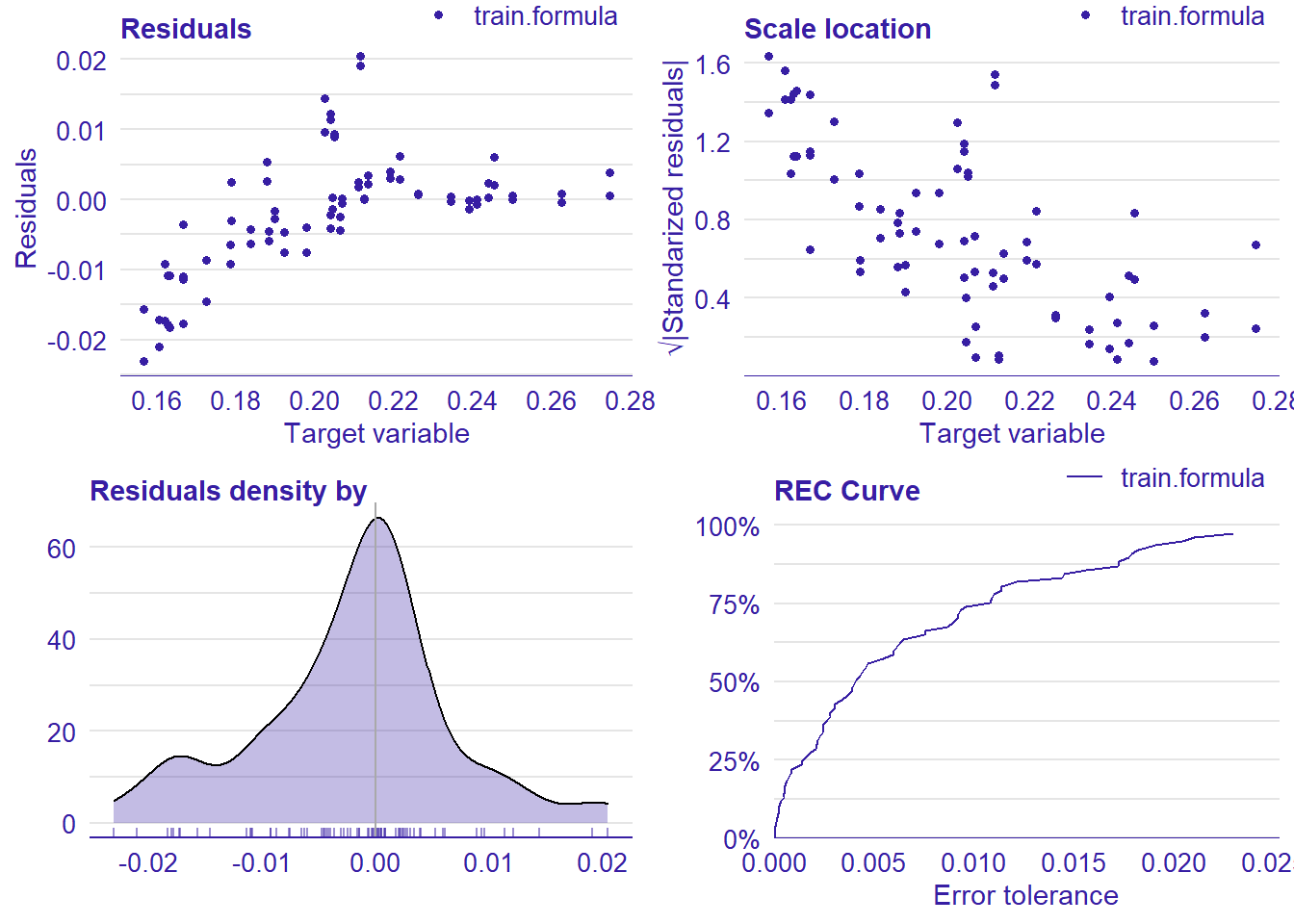Figure 26: Data fit with Random Forest, Year 2014 - 2018

## TableGrob (2 x 2) "arrange": 4 grobs
##   z     cells    name           grob
## 1 1 (1-1,1-1) arrange gtable[layout]
## 2 2 (1-1,2-2) arrange gtable[layout]
## 3 3 (2-2,1-1) arrange gtable[layout]
## 4 4 (2-2,2-2) arrange gtable[layout]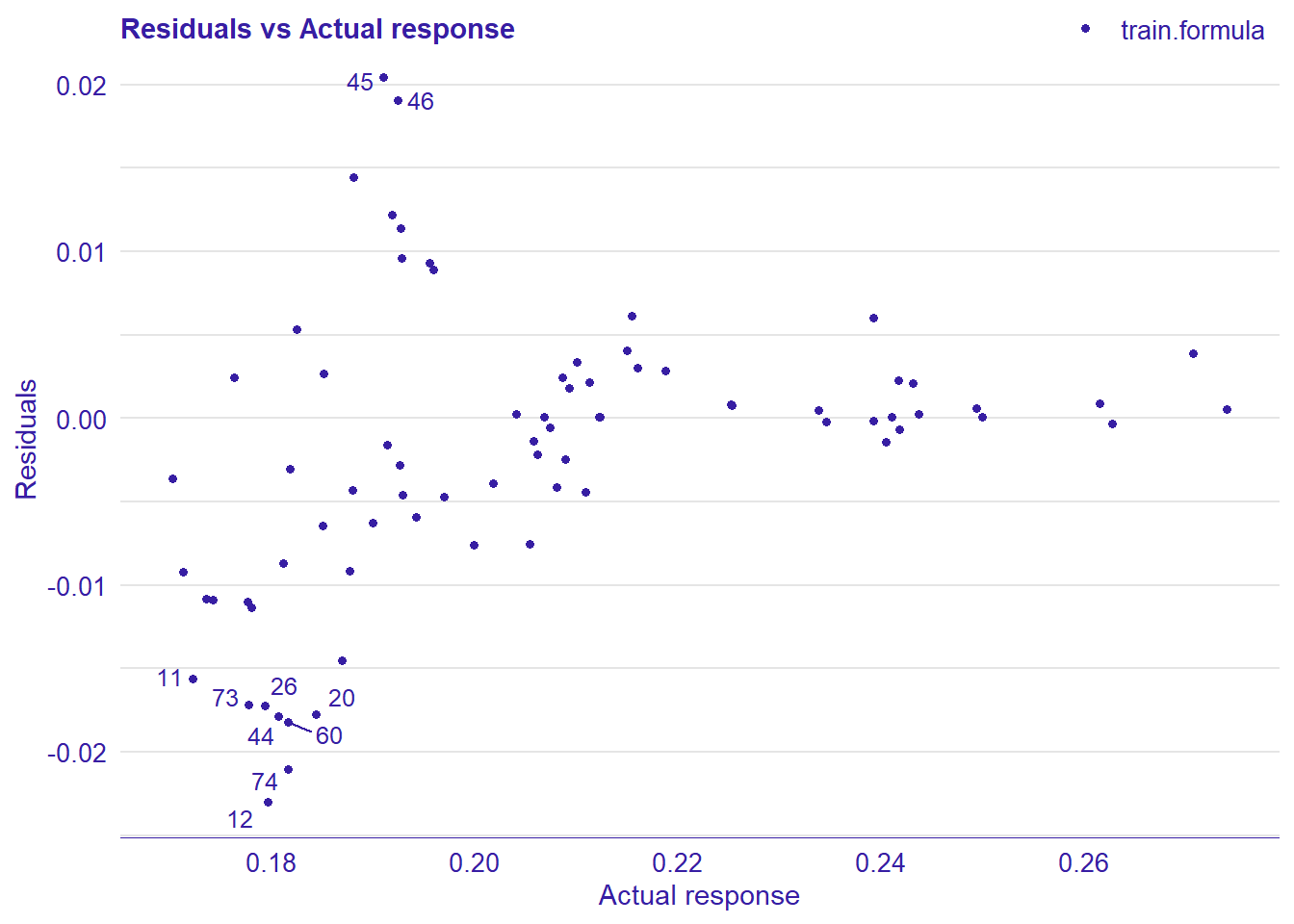Figure 27: Data fit with Random Forest, Year 2014 - 2018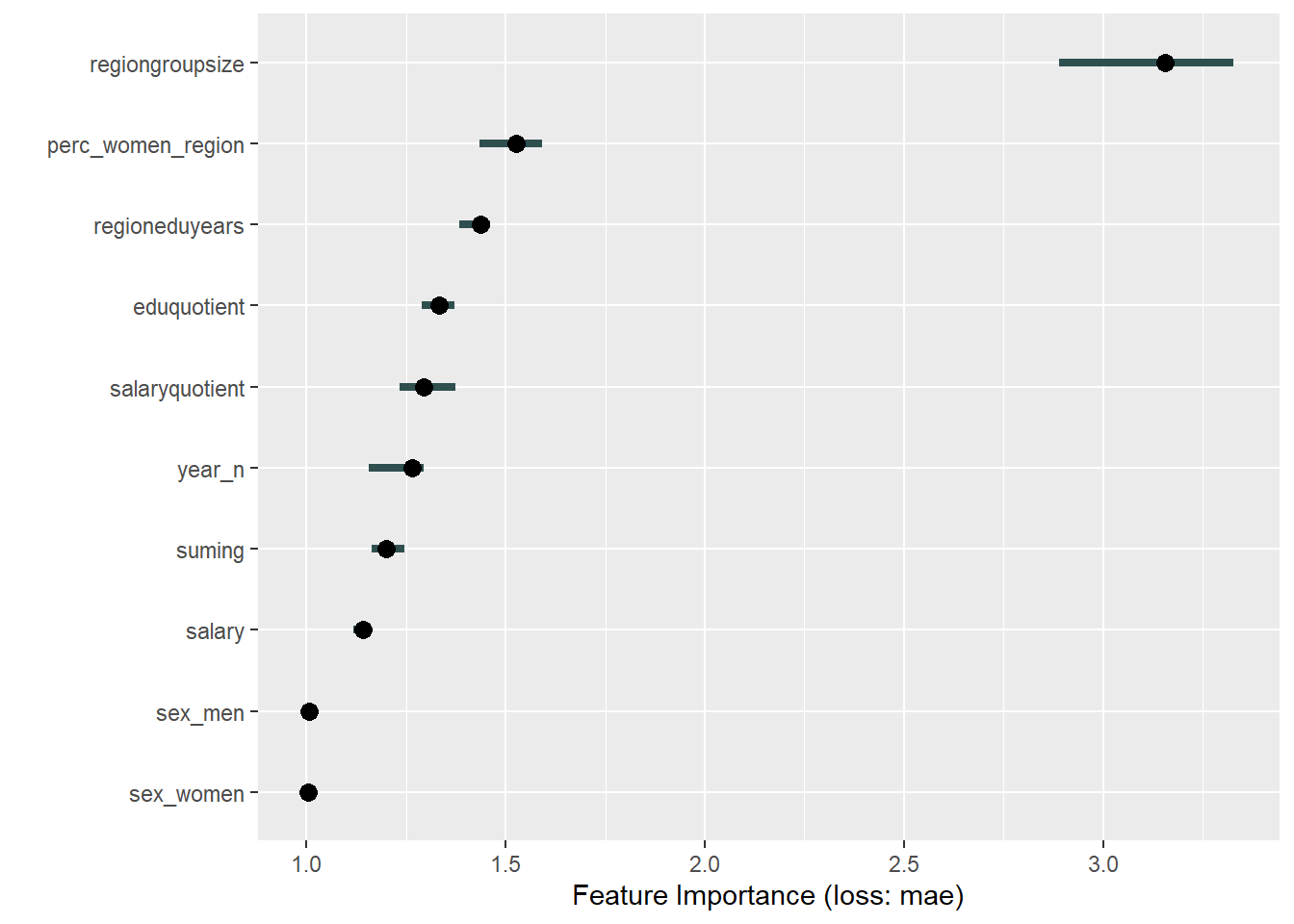Figure 28: Data fit with Random Forest, Year 2014 - 2018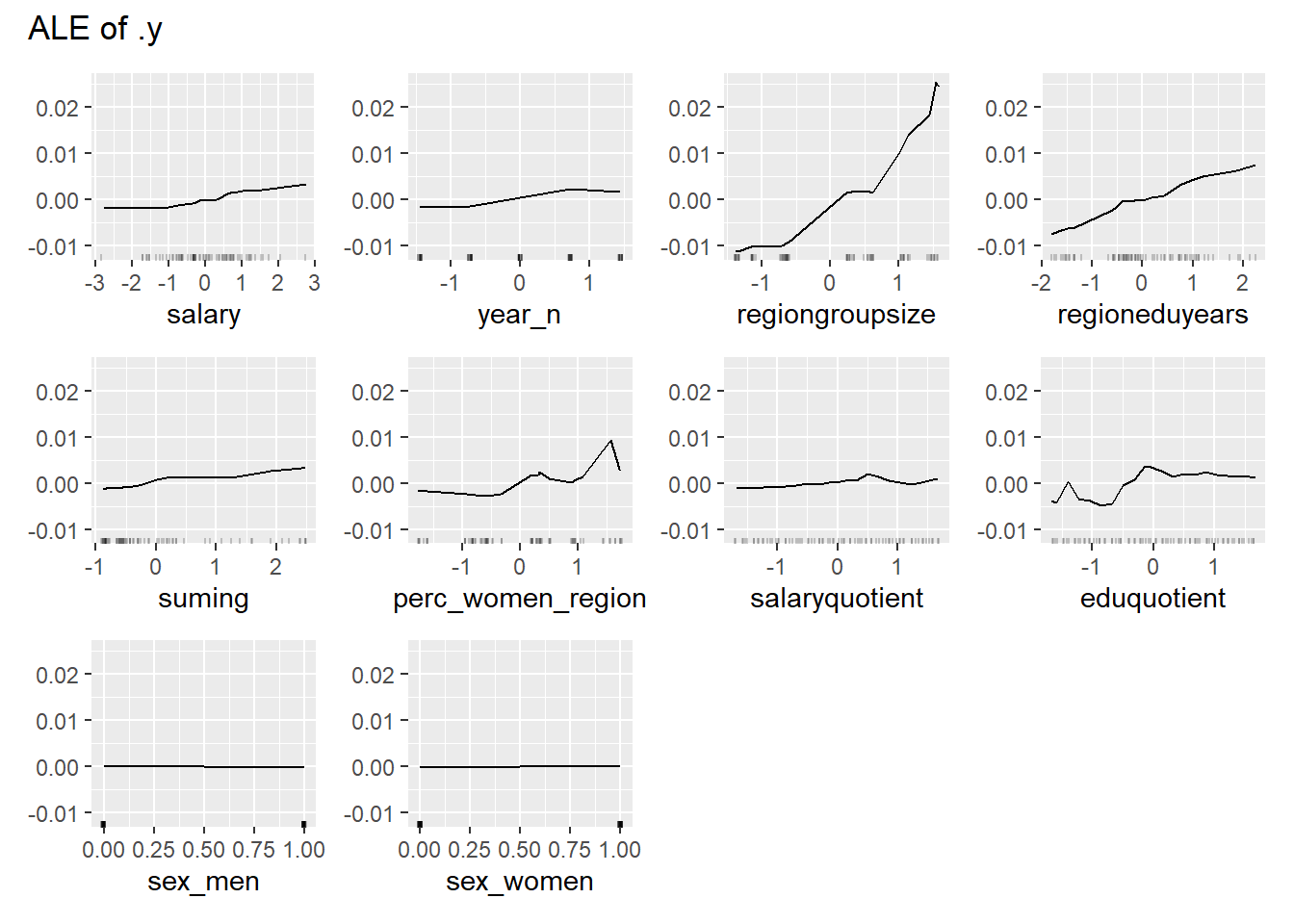Figure 29: Data fit with Random Forest, Year 2014 - 2018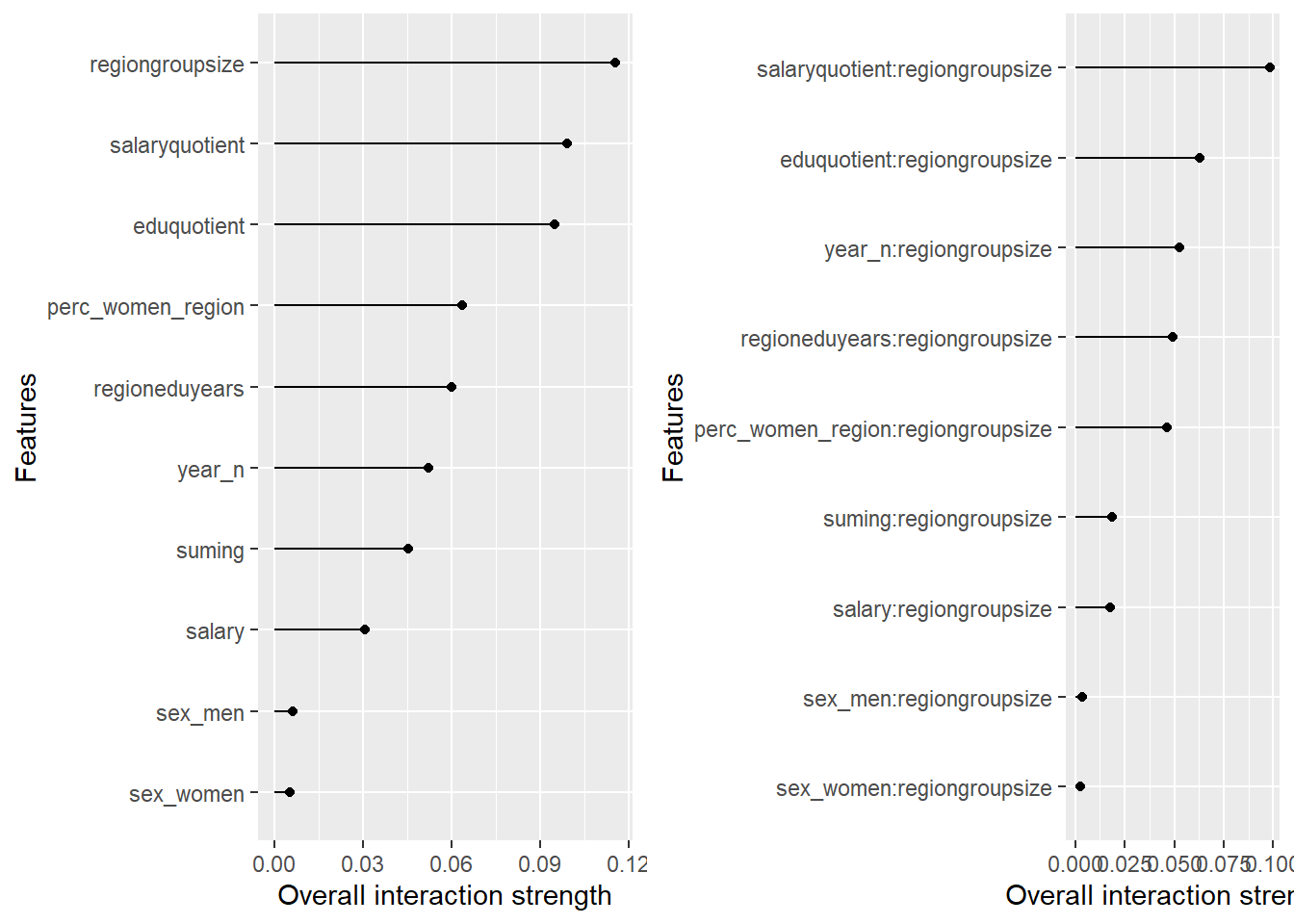Figure 30: Data fit with Random Forest, Year 2014 - 2018

## TableGrob (1 x 2) "arrange": 2 grobs
##   z     cells    name           grob
## 1 1 (1-1,1-1) arrange gtable[layout]
## 2 2 (1-1,2-2) arrange gtable[layout]

Data fit with Gradient Boosting Machine

tr <- trainControl(method = "repeatedcv", number = 10, repeats = 5)

tg <- expand.grid(shrinkage = seq(0.1),
interaction.depth = c(3),
n.minobsinnode = c(10),
n.trees = c(100))

set.seed(123)
model <- caret::train(
perc_women_eng_region ~ .,
data = tbnum,
weights = tbnum_weights,
method = "gbm",
trControl = tr,
tuneGrid = tg,
verbose = FALSE)

plot_model(model)
##
##  "Model RMSE:  0.00352511569538881"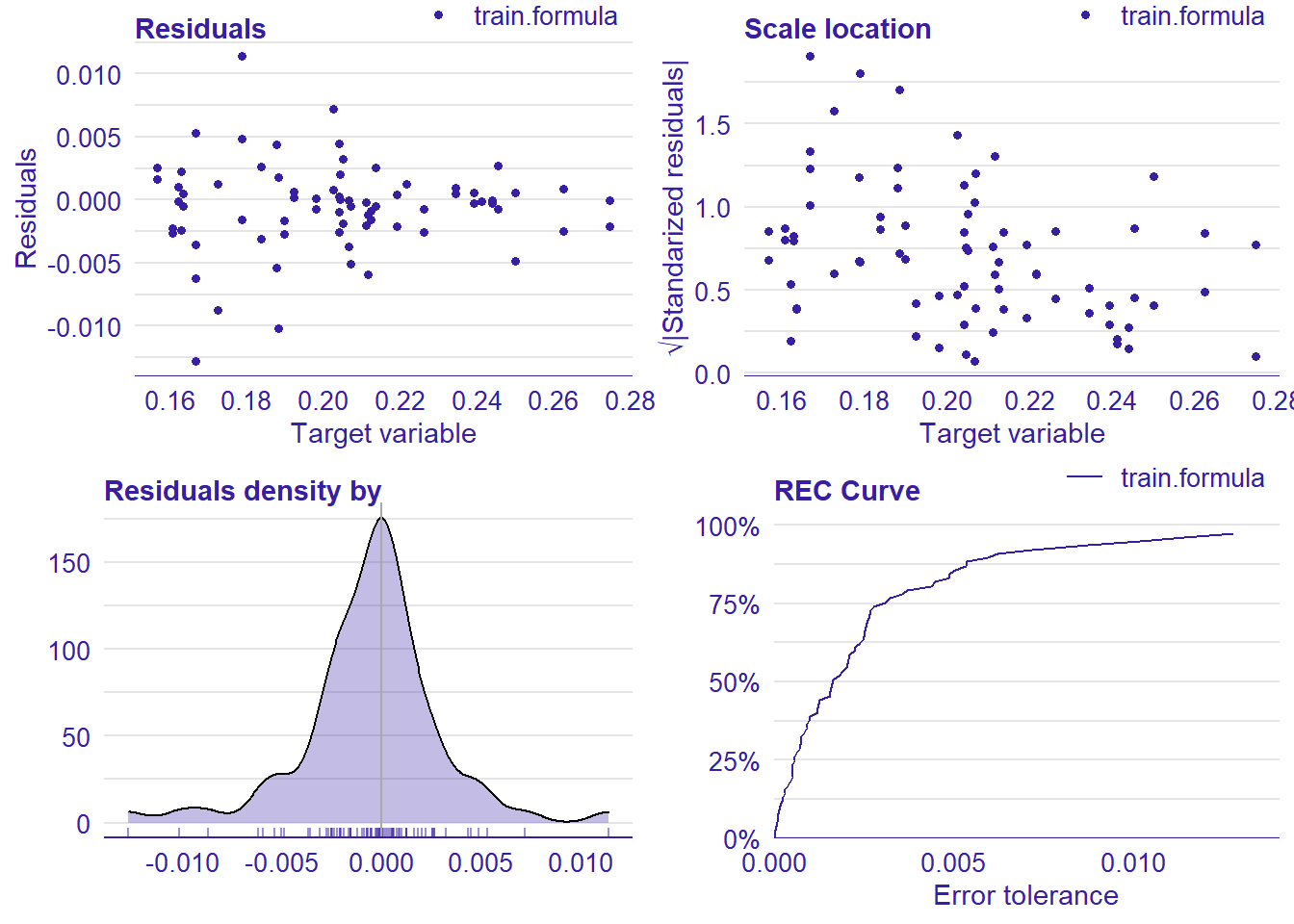Figure 31: Data fit with Gradient Boosting Machine, Year 2014 - 2018

## TableGrob (2 x 2) "arrange": 4 grobs
##   z     cells    name           grob
## 1 1 (1-1,1-1) arrange gtable[layout]
## 2 2 (1-1,2-2) arrange gtable[layout]
## 3 3 (2-2,1-1) arrange gtable[layout]
## 4 4 (2-2,2-2) arrange gtable[layout]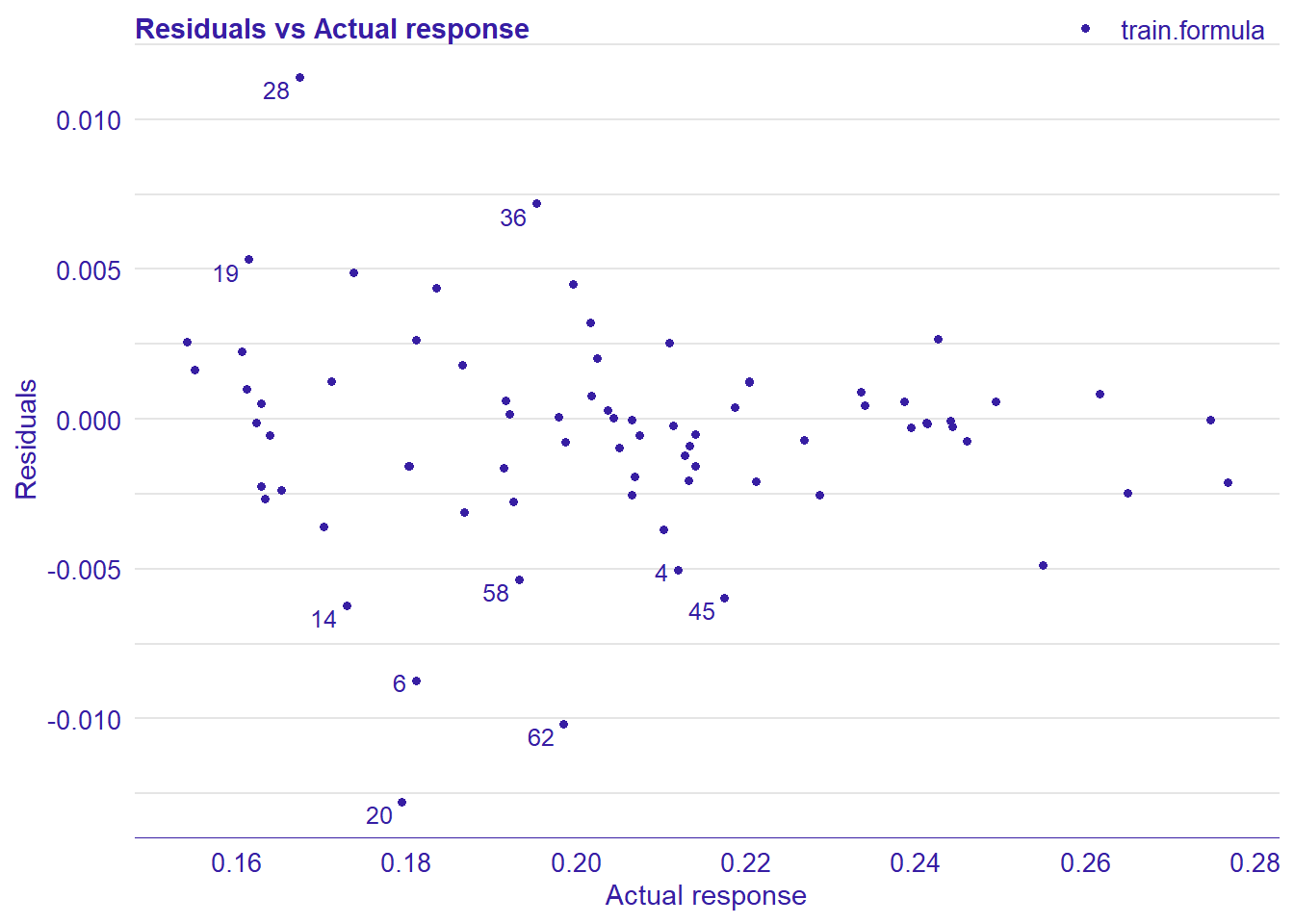Figure 32: Data fit with Gradient Boosting Machine, Year 2014 - 2018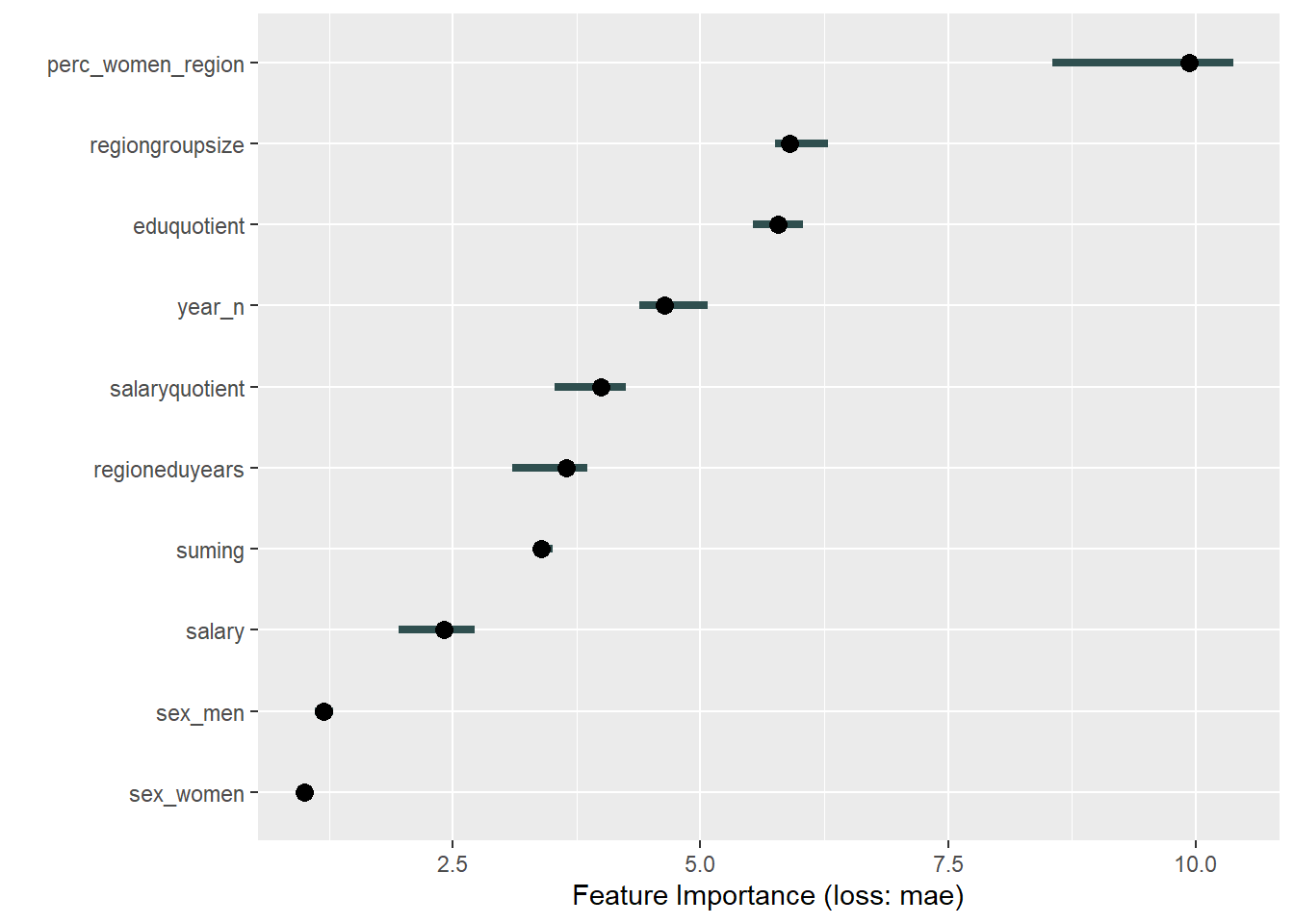Figure 33: Data fit with Gradient Boosting Machine, Year 2014 - 2018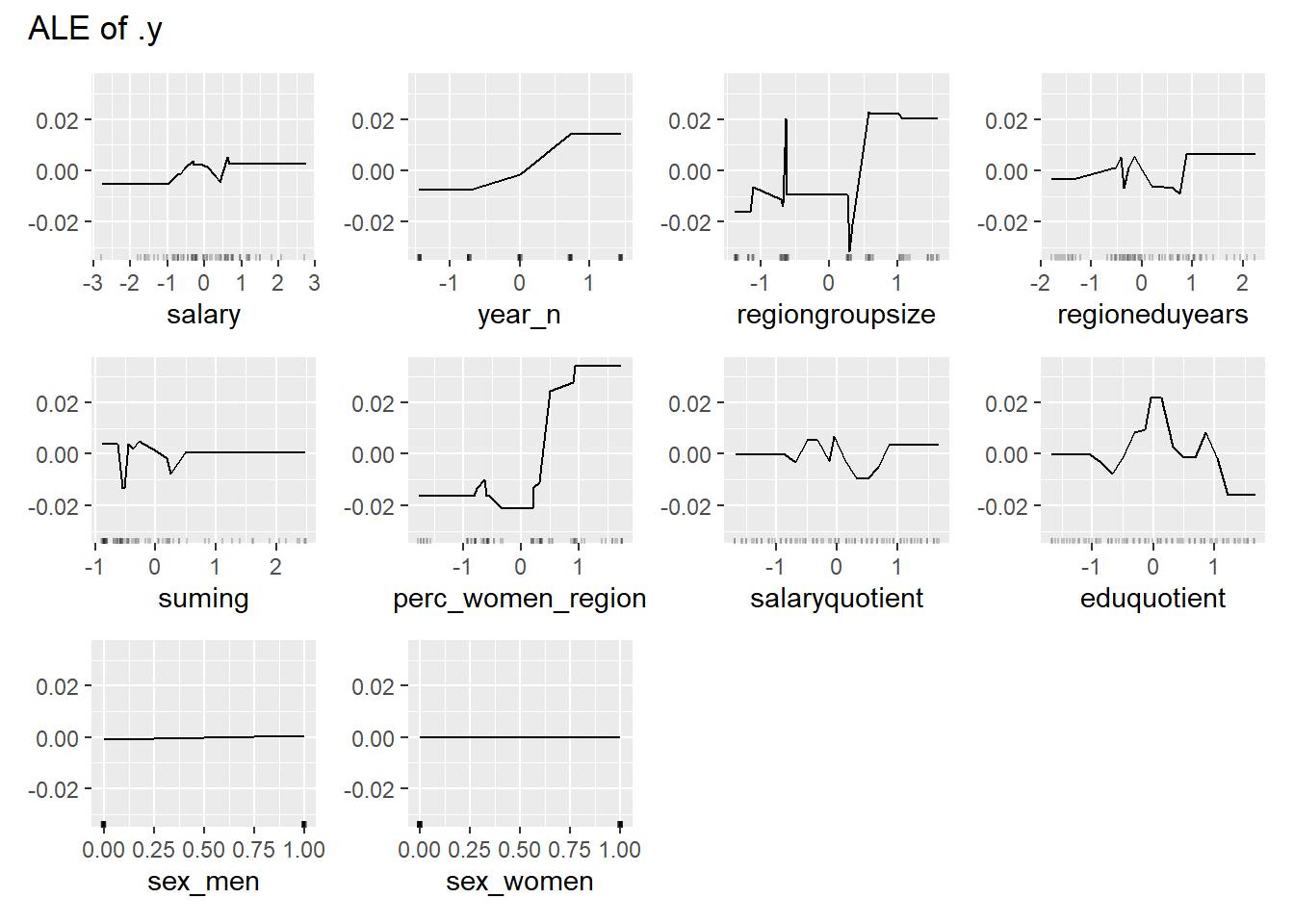Figure 34: Data fit with Gradient Boosting Machine, Year 2014 - 2018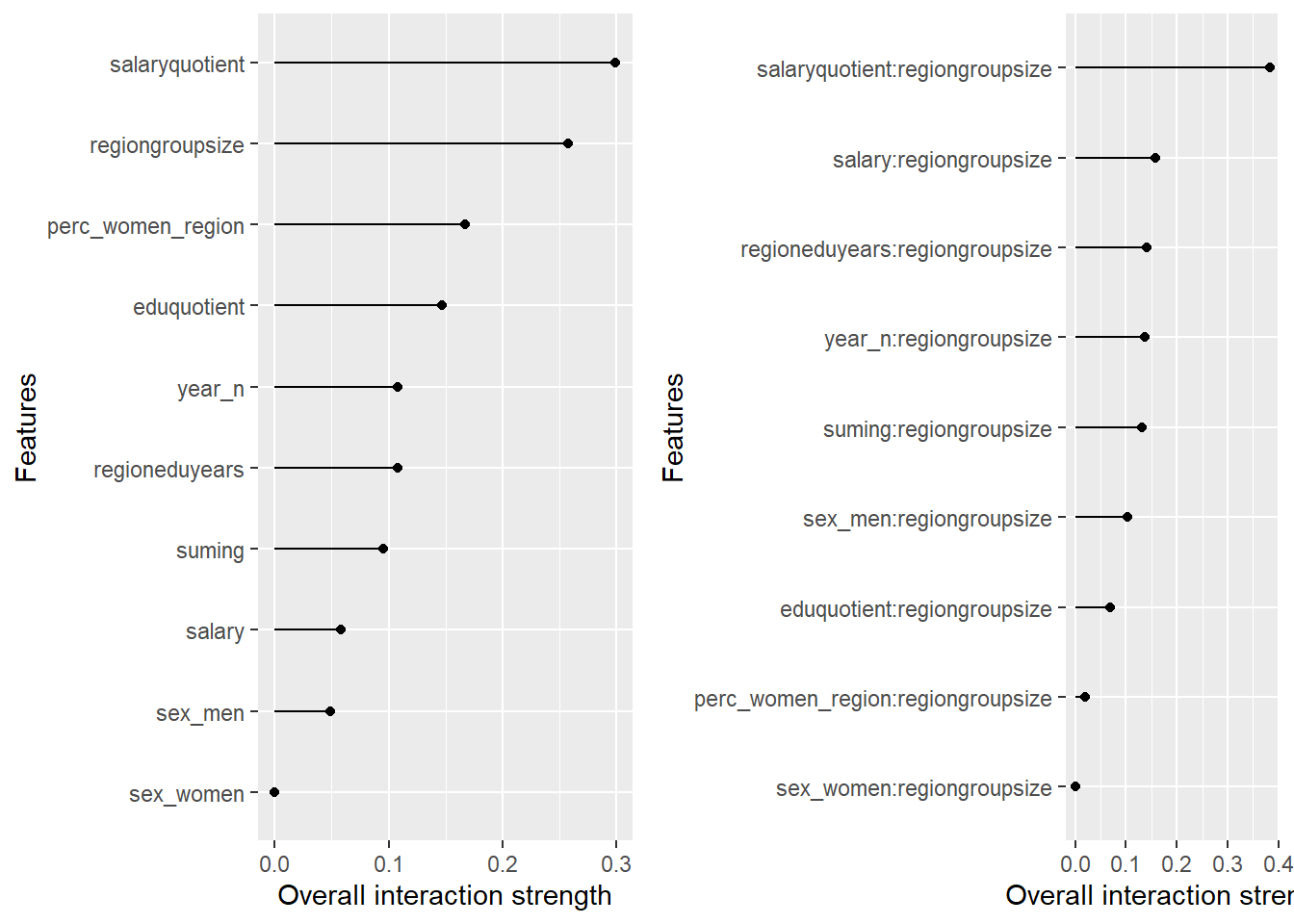Figure 35: Data fit with Gradient Boosting Machine, Year 2014 - 2018

## TableGrob (1 x 2) "arrange": 2 grobs
##   z     cells    name           grob
## 1 1 (1-1,1-1) arrange gtable[layout]
## 2 2 (1-1,2-2) arrange gtable[layout]

Data fit with Deep Learning

rctrl1 <- trainControl(method = "cv", number = 3, returnResamp = "all")

set.seed(123)  # for reproducibility
model <- caret::train(
perc_women_eng_region ~ .,
data = tbnum,
weights = tbnum_weights,
method = "neuralnet",
trControl = rctrl1,
tuneGrid = data.frame(layer1 = 2:20, layer2 = 2:20, layer3 = 2:20),
rep = 3,
threshold = 0.0001,
preProc = c("center", "scale"))

plot_model(model)
##
##  "Model RMSE:  0.00924842751296535"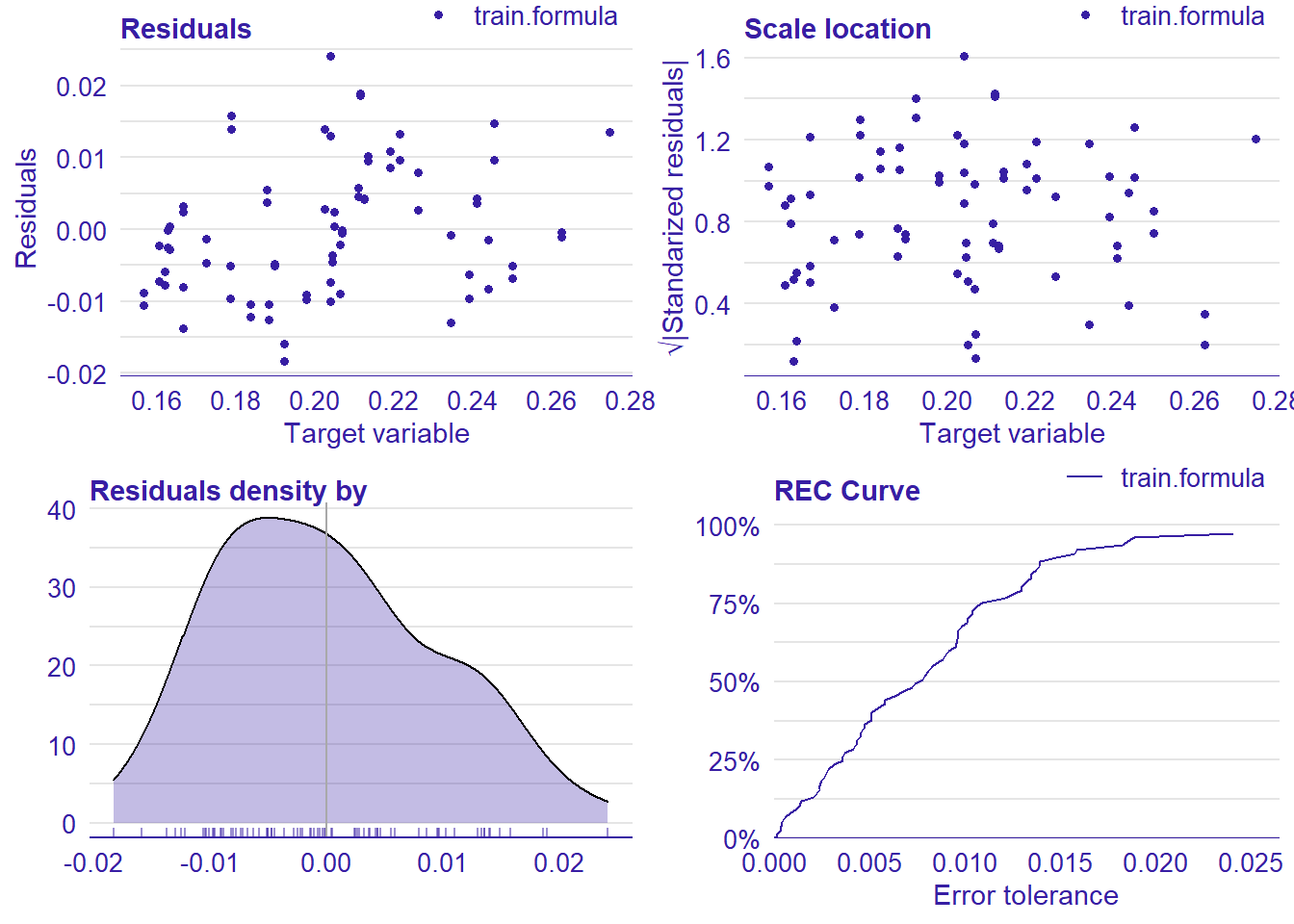Figure 36: Data fit with Deep Learning, Year 2014 - 2018

## TableGrob (2 x 2) "arrange": 4 grobs
##   z     cells    name           grob
## 1 1 (1-1,1-1) arrange gtable[layout]
## 2 2 (1-1,2-2) arrange gtable[layout]
## 3 3 (2-2,1-1) arrange gtable[layout]
## 4 4 (2-2,2-2) arrange gtable[layout]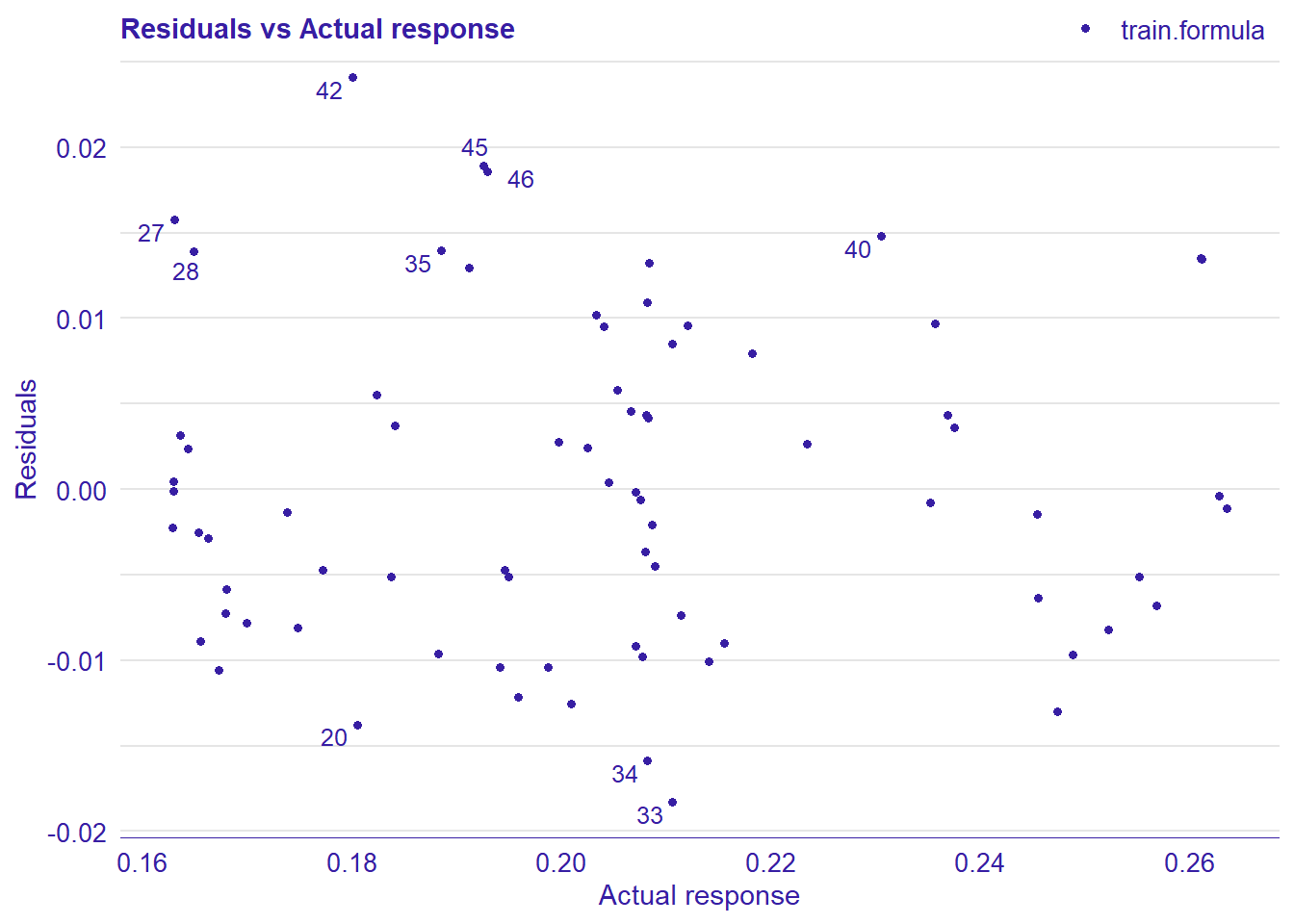Figure 37: Data fit with Deep Learning, Year 2014 - 2018Figure 38: Data fit with Deep Learning, Year 2014 - 2018Figure 39: Data fit with Deep Learning, Year 2014 - 2018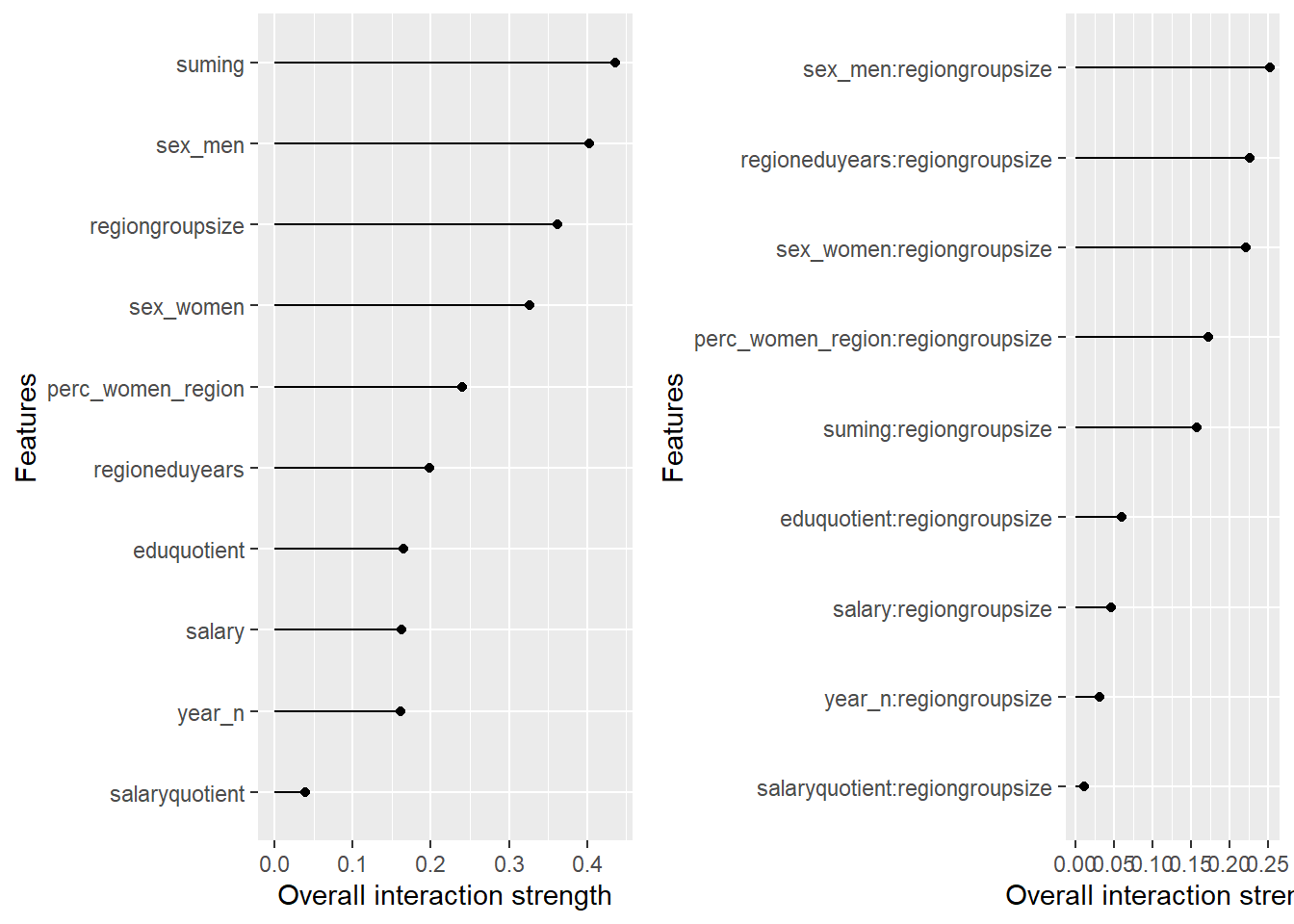Figure 40: Data fit with Deep Learning, Year 2014 - 2018

## TableGrob (1 x 2) "arrange": 2 grobs
##   z     cells    name           grob
## 1 1 (1-1,1-1) arrange gtable[layout]
## 2 2 (1-1,2-2) arrange gtable[layout]

Data fit with Support Vector Machine

set.seed(123)  # for reproducibility
model <- caret::train(
perc_women_eng_region ~ .,
data = tbnum,
weights = tbnum_weights,
preProcess = c("center", "scale"),
trControl = trainControl(method = "cv", number = 10),
tuneLength = 10
)

plot_model(model)
##
##  "Model RMSE:  0.00848330404577974"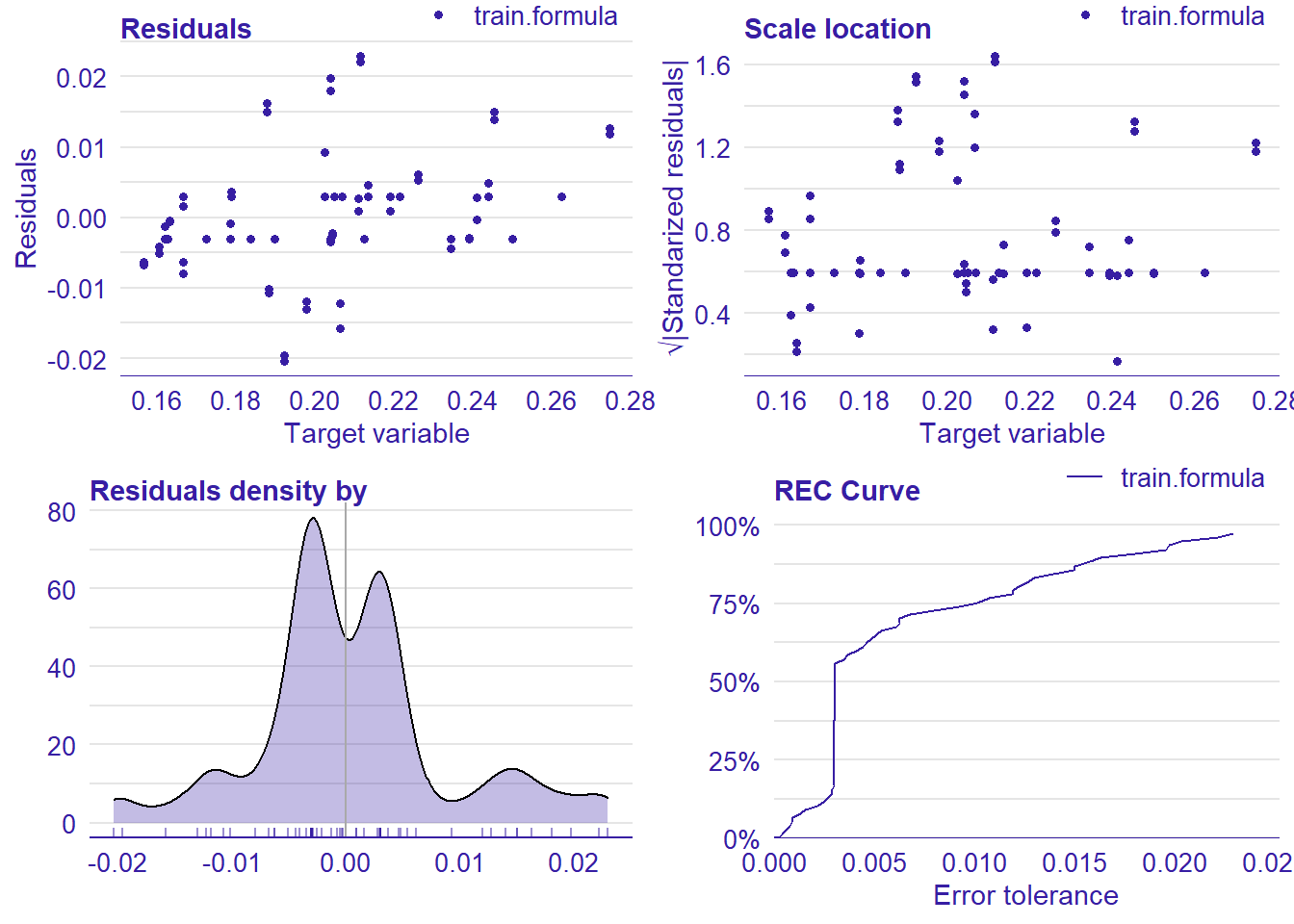Figure 41: Data fit with Support Vector Machine, Year 2014 - 2018

## TableGrob (2 x 2) "arrange": 4 grobs
##   z     cells    name           grob
## 1 1 (1-1,1-1) arrange gtable[layout]
## 2 2 (1-1,2-2) arrange gtable[layout]
## 3 3 (2-2,1-1) arrange gtable[layout]
## 4 4 (2-2,2-2) arrange gtable[layout]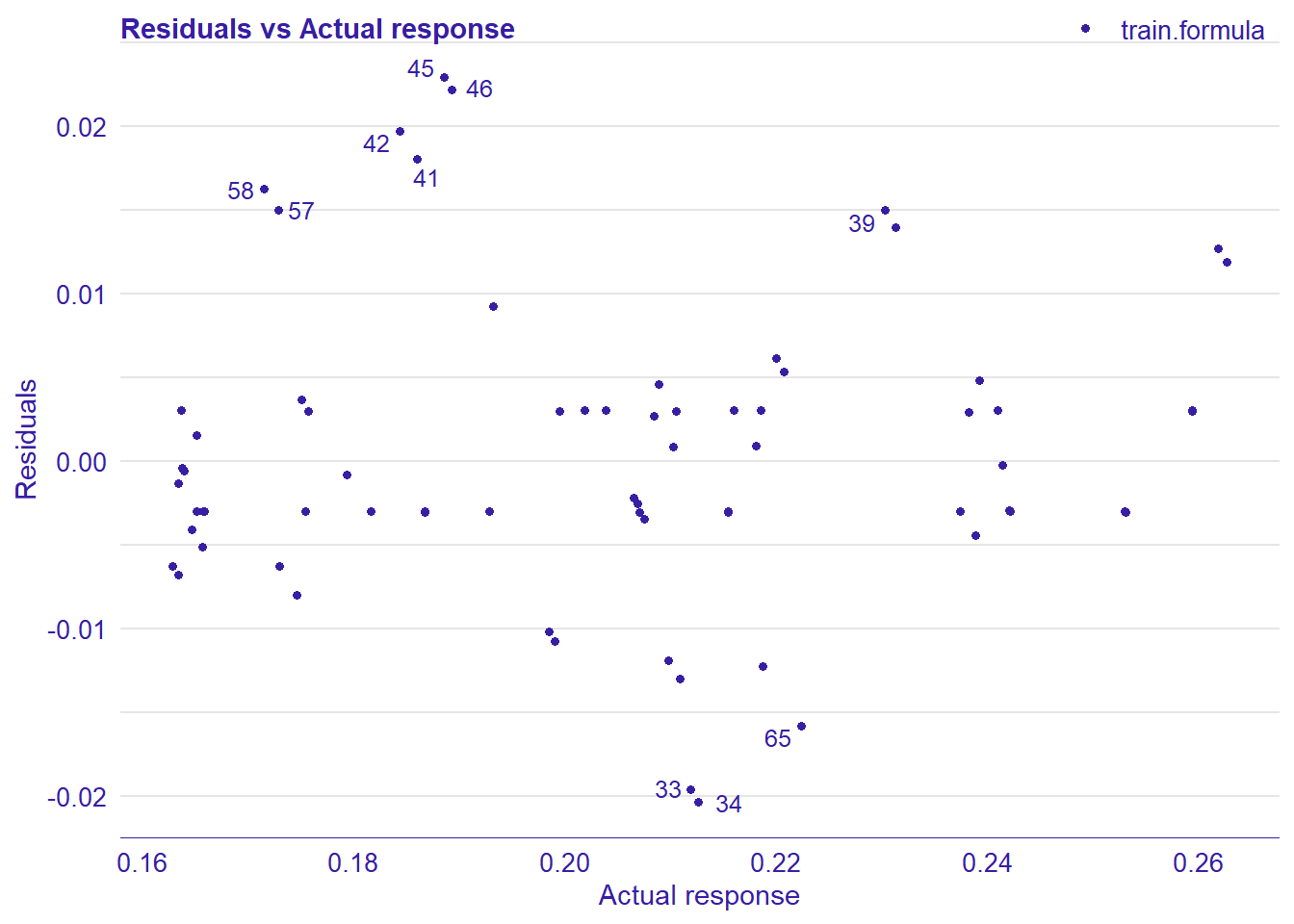Figure 42: Data fit with Support Vector Machine, Year 2014 - 2018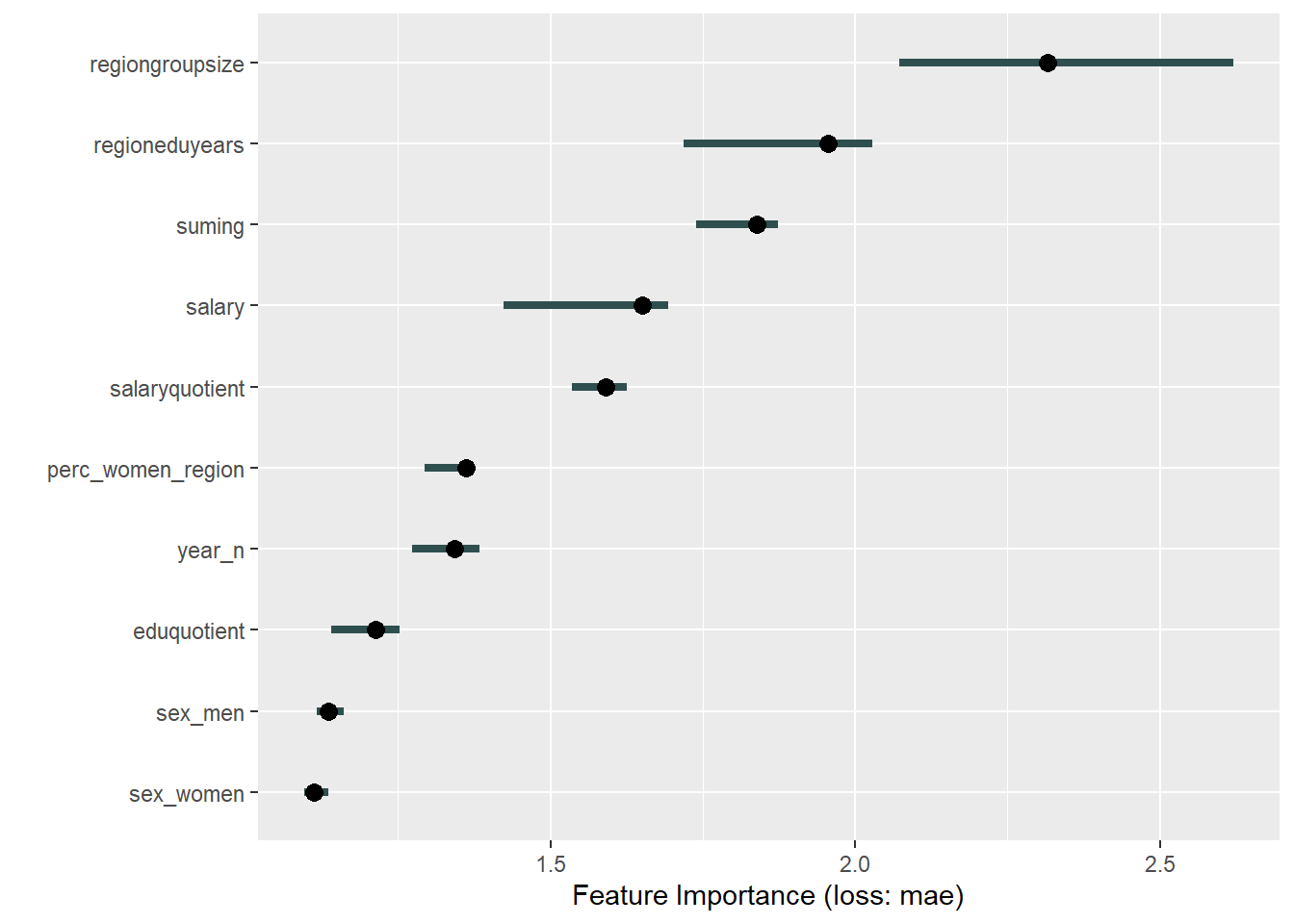Figure 43: Data fit with Support Vector Machine, Year 2014 - 2018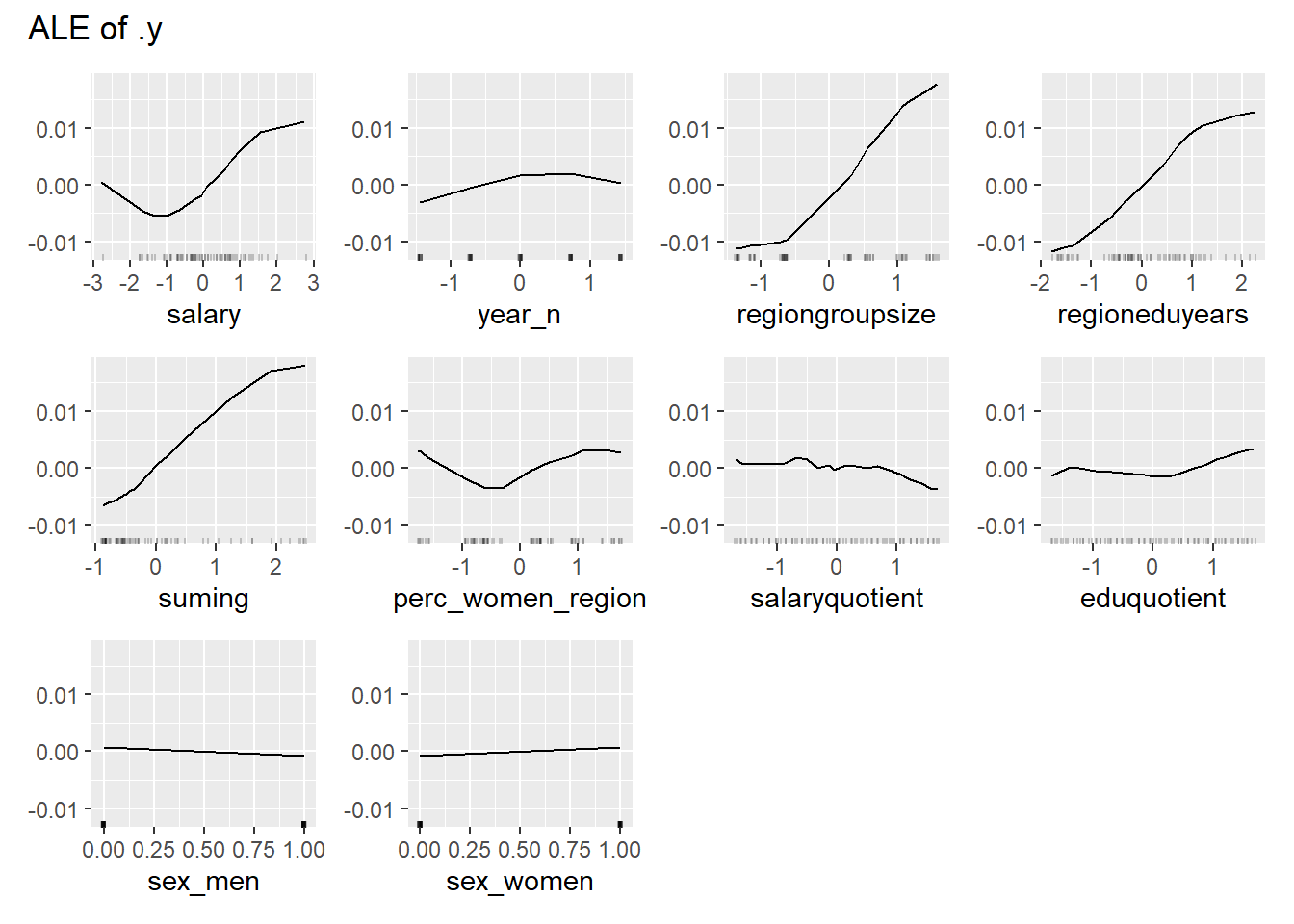Figure 44: Data fit with Support Vector Machine, Year 2014 - 2018Figure 45: Data fit with Support Vector Machine, Year 2014 - 2018

## TableGrob (1 x 2) "arrange": 2 grobs
##   z     cells    name           grob
## 1 1 (1-1,1-1) arrange gtable[layout]
## 2 2 (1-1,2-2) arrange gtable[layout]